# JPWO2005111635A1 - Method and apparatus for measuring electric circuit parameters - Google Patents

## Info

Publication number
JPWO2005111635A1
JPWO2005111635A1 JP2006513567A JP2006513567A JPWO2005111635A1 JP WO2005111635 A1 JPWO2005111635 A1 JP WO2005111635A1 JP 2006513567 A JP2006513567 A JP 2006513567A JP 2006513567 A JP2006513567 A JP 2006513567A JP WO2005111635 A1 JPWO2005111635 A1 JP WO2005111635A1
Authority
JP
Japan
Prior art keywords
terminal
measuring
measurement
line
circuit
Prior art date
Legal status (The legal status is an assumption and is not a legal conclusion. Google has not performed a legal analysis and makes no representation as to the accuracy of the status listed.)
Granted
Application number
JP2006513567A
Other languages
Japanese (ja)
Inventor

Original Assignee

Priority date (The priority date is an assumption and is not a legal conclusion. Google has not performed a legal analysis and makes no representation as to the accuracy of the date listed.)
Filing date
Publication date
Priority to JP2004144809 priority Critical
Priority to JP2004144809 priority
Application filed by 松下電器産業株式会社 filed Critical 松下電器産業株式会社
Priority to PCT/JP2005/008782 priority patent/WO2005111635A1/en
Publication of JPWO2005111635A1 publication Critical patent/JPWO2005111635A1/en
Granted legal-status Critical Current

• 239000000758 substrates Substances 0.000 claims description 54
• 238000004364 calculation methods Methods 0.000 claims description 28
• 239000003990 capacitor Substances 0.000 claims description 7
• 238000000691 measurement method Methods 0.000 description 46
• 239000011159 matrix materials Substances 0.000 description 44
• 230000005540 biological transmission Effects 0.000 description 32
• 238000010586 diagrams Methods 0.000 description 32
• 239000000203 mixtures Substances 0.000 description 28
• 238000006243 chemical reactions Methods 0.000 description 18
• 230000014509 gene expression Effects 0.000 description 18
• 229910052751 metals Inorganic materials 0.000 description 14
• 239000002184 metals Substances 0.000 description 14
• 230000000875 corresponding Effects 0.000 description 12
• 239000007787 solids Substances 0.000 description 12
• 280000409857 Coaxial Cable companies 0.000 description 10
• 230000003071 parasitic Effects 0.000 description 10
• 238000004088 simulation Methods 0.000 description 10
• 239000003989 dielectric materials Substances 0.000 description 8
• 230000001965 increased Effects 0.000 description 6
• 239000004020 conductors Substances 0.000 description 4
• 230000000694 effects Effects 0.000 description 4
• 230000001939 inductive effects Effects 0.000 description 4
• 238000000034 methods Methods 0.000 description 4
• 230000037361 pathway Effects 0.000 description 4
• 238000000926 separation method Methods 0.000 description 4
• 229910001218 Gallium arsenide Inorganic materials 0.000 description 2
• 280000539100 Infinity as companies 0.000 description 2
• 210000001503 Joints Anatomy 0.000 description 2
• 241000218641 Pinaceae Species 0.000 description 2
• 230000002411 adverse Effects 0.000 description 2
• 239000010410 layers Substances 0.000 description 2
• 239000004973 liquid crystal related substances Substances 0.000 description 2
• 239000000463 materials Substances 0.000 description 2
• 230000035945 sensitivity Effects 0.000 description 2

## Images

•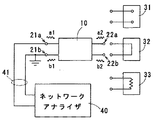•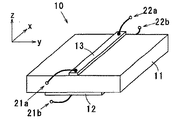•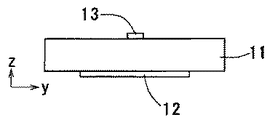•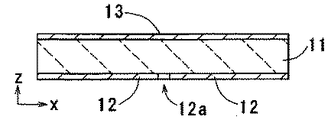•••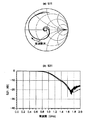•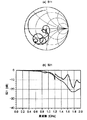•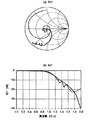•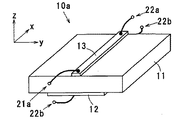•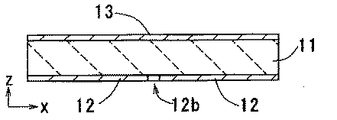•••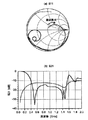•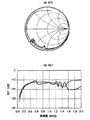•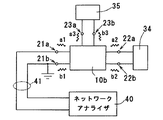•••••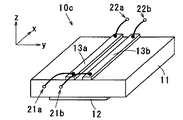•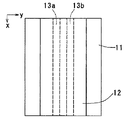•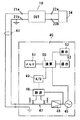•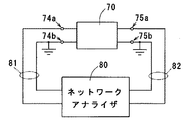••••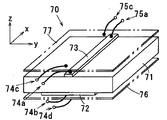•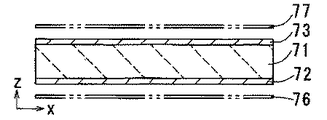•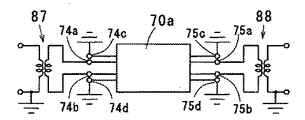•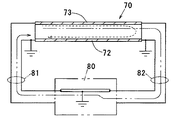•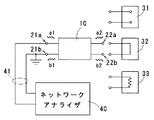••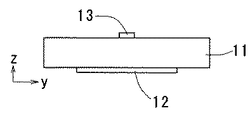•••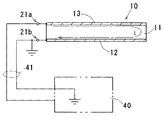•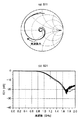•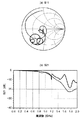•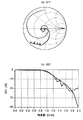••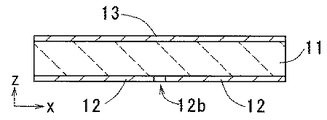•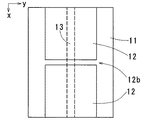••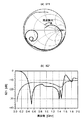•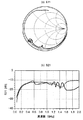•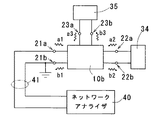•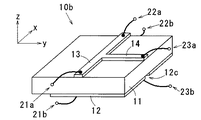••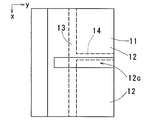•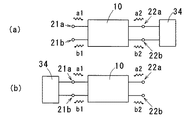•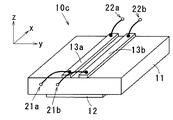•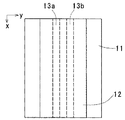••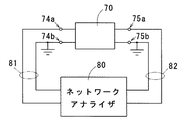•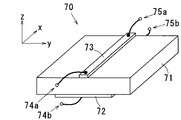•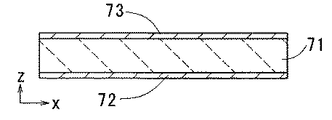•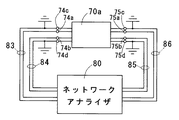••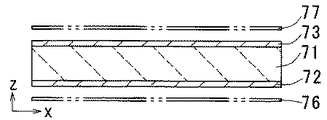•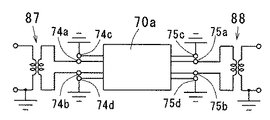•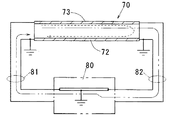## Classifications

• GPHYSICS
• G01MEASURING; TESTING
• G01RMEASURING ELECTRIC VARIABLES; MEASURING MAGNETIC VARIABLES
• G01R27/00Arrangements for measuring resistance, reactance, impedance, or electric characteristics derived therefrom
• G01R27/28Measuring attenuation, gain, phase shift or derived characteristics of electric four pole networks, i.e. two-port networks using network analysers Measuring transient response

## Abstract

The method for measuring the electric circuit parameter includes the step of measuring the reflection characteristic α1 relating to the other terminal in a state where one of the first terminal and the second terminal of the two-terminal network is terminated with the termination impedance Z1, and the first terminal And measuring one of the first terminal and the second terminal, the step of measuring the reflection characteristic α2 related to the other terminal in a state where either one of the second terminal and the second terminal is terminated with a termination impedance Z2 different from the termination impedance Z1. In a state where the terminal impedance Z3 is different from the terminal impedances Z1 and Z2, the step of measuring the reflection characteristic α3 related to the other terminal, and the electrical characteristics of the two-terminal network based on the obtained reflection characteristics α1, α2, and α3. Calculating circuit parameters. With such a configuration, even a device under measurement having a weak ground can measure electric circuit parameters such as S parameters and Z parameters with high accuracy and at low cost.

## Description

The present invention evaluates signal quality (SI: Signal Integrity), power supply-ground line quality (PI) by measuring electric circuit parameters of an N-terminal network (N is an integer of 2 or more) having a plurality of input / output terminals. The present invention relates to a method and apparatus for measuring electric circuit parameters that contribute to evaluation of power integrity (EMI), evaluation of unnecessary electromagnetic radiation (EMI), and the like.

Mobile electronic devices typified by mobile phones, laptop computers, digital cameras, etc., and digital home appliances typified by digital televisions and DVDs (Digital Video Discs) have rapidly advanced in their performance and functionality. Therefore, higher signal processing speed and signal transmission rate are required.

Since it is difficult to transmit high-speed digital signals only by simple wiring design, evaluation of signal quality (SI) is important. In addition, if high frequency noise is superimposed on the power supply or the ground itself, it may adversely affect the operation of an electronic component such as an LSI. Therefore, it is important to evaluate the quality (PI) of the power supply-ground line. In addition, if the designed ground is not strong enough and a return current path cannot be secured, current in the same phase will flow through the signal line and ground, causing unnecessary radiation (EMI) and causing interference with TVs and radios. Or other electronic devices may malfunction. Problems common to such SI, PI, and EMI include a) countermeasures for increasing the signal speed and b) countermeasures for constrained ground design.

For example, with regard to SI, the effects of parasitic impedances associated with wiring and the reflection of signals that occur at the joints of wiring such as input parts and vias to electronic components were not considered as a problem at low transmission speeds. It becomes important as the signal speed increases. As a countermeasure, a wiring design that takes into account impedance by providing a strong ground with a large area can be considered. However, in reality, it is extremely difficult to provide a strong ground having a large area due to constraints such as downsizing, high density, and cost reduction (for example, reduction in the number of layers of a multilayer substrate).

With respect to PI, there is a problem that the ground potential called ground bounce changes in an alternating manner. One of the factors is that the power supply-ground line has a finite impedance (for example, inductive impedance), and voltage fluctuation occurs when an AC signal flows. If the finite impedance is inductive, the value is L, and the current value is I, the ground potential fluctuation can be expressed by L × (dI / dt), which increases with higher speed and higher frequency. A weak ground has a large impedance value, which promotes the PI problem.

Similarly to the above, the main factor of EMI is that the current return path cannot be secured well, and is closely related to the strength and frequency of the ground.

In order to measure and evaluate SI, PI, and EMI as described above, a measurement method for measuring and evaluating a transmission line having a weak ground and a power-ground line at high speed and high frequency is necessary.

The following Patent Document 1 relates to a near electromagnetic field measurement technique, and is radiated from an electric circuit including a transmission line having a weak ground, a power source-ground, etc. by bringing an electromagnetic field probe close to an electronic component or a transmission line. The amount of EMI is measured. In particular, when the power supply-ground line is approached, indirect PI evaluation is possible.

Next, there is a method of directly measuring the impedance of the transmission line.

FIG. 17 is a block diagram showing an example of a conventional electric circuit parameter measurement system. Here, a two-terminal circuit 70 having a pair of input terminals 74a and 74b and a pair of output terminals 75a and 75b is used as a device under test (DUT: Device Under Test).

FIG. 18A is a perspective view showing an example of the two-terminal circuit 70, and FIG. 18B is a cross-sectional view along the longitudinal direction of the signal strip line 73. The two-terminal circuit 70 includes an electrically insulating substrate 71, a ground pattern 72 provided on the lower surface of the substrate 71, and a signal strip line 73 provided on the upper surface of the substrate 71. The input terminal 74 a is connected to one end of the signal strip line 73, and the input terminal 74 b is connected to one end of the ground pattern 72. The output terminal 75 a is connected to the other end of the signal strip line 73, and the output terminal 75 b is connected to the other end of the ground pattern 72.

As shown in FIG. 17, the input terminals 74 a and 74 b of the two-terminal circuit 70 are connected to the network analyzer 80 via the signal cable 81, and the output terminals 75 a and 75 b are connected to the network analyzer 80 via the signal cable 82. Connected. The input terminal 74b and the output terminal 75b are grounded to maintain the ground potential.

A vector network analyzer (VNA) 80 supplies a reference signal to the device under measurement, and measures the amplitude and phase of the signal that has passed through the device under measurement and the amplitude and phase of the signal reflected from the device under measurement. Then, the line characteristic of the device under measurement, for example, the S parameter is measured. Here, while the reference signal is supplied to the input terminals 74a and 74b via the signal cable 81, the passing signal of the two-terminal circuit 70 output from the output terminals 75a and 75b is measured via the signal cable 82. By measuring the reflected signal of the two-terminal circuit 70 returning from the input terminals 74a and 74b via the signal cable 81, the S parameter of the 2 × 2 matrix is measured for the two-terminal circuit 70.

FIG. 19 is a block diagram showing another example of a conventional electric circuit parameter measurement system. Here, as the device under test (DUT), not only the signal strip line 73 in the two-terminal circuit 70 shown in FIG. 18 but also the ground pattern 72 is regarded as a kind of signal line, and a pair of input terminals 74a and 74c, The measurement is performed as a four-terminal circuit 70a having a pair of output terminals 75b and 75d, a pair of output terminals 75a and 75c, and a pair of output terminals 75b and 75d.

20A is a perspective view showing an example of the four-terminal circuit 70 a, and FIG. 20B is a cross-sectional view along the longitudinal direction of the signal strip line 73. The four-terminal circuit 70a includes two dummy metal plates 76 and 77 added to the two-terminal circuit 70 shown in FIG. 18, and is arranged in parallel above and below the substrate 71, respectively.

The input terminal 74a is connected to one end of the signal strip line 73, the input terminal 74b is connected to one end of the ground pattern 72, and the input terminals 74c and 74d are connected to one end of the dummy metal plates 76 and 77, respectively. The output terminal 75a is connected to the other end of the signal strip line 73, the output terminal 75b is connected to the other end of the ground pattern 72, and the output terminals 75c and 75d are connected to the other ends of the dummy metal plates 76 and 77, respectively.

As shown in FIG. 19, the input terminals 74a and 74c of the four-terminal circuit 70a are routed through the signal cable 83, the input terminals 74b and 74d are routed through the signal cable 84, and the output terminals 75a and 75c are routed through the signal cable 85. The output terminals 75b and 75d are connected to the network analyzer 80 via the signal cable 85. Therefore, when a coaxial cable is used as each of the signal cables 83 to 86, the input terminal 74b and the output terminal 75b of the ground pattern 72 are also connected by a core wire. The input terminals 74c and 74d and the output terminals 75c and 75d are grounded to maintain the ground potential.

When the device under test is configured as a four-terminal circuit 70a in this way, S parameters of a 4 × 4 matrix are measured.

FIG. 21 is a circuit diagram showing an example of a conversion circuit from a 4-terminal circuit to a 2-terminal circuit. An input-side balun 87 is connected to the input terminals 74a and 74b of the 4-terminal circuit 70a, an output-side balun 88 is connected to the output terminals 75a and 75b, and the input terminals 74c and 74d and the output terminals 75c and 75d are grounded. Yes. By arranging these baluns 87 and 88 on the input / output side, four unbalanced signals can be converted into two balanced signals.

For example, when such a conversion circuit is configured on a circuit simulator, the S parameter of the 4 × 4 matrix of the 4-terminal circuit 70a obtained by the above-described measurement is a 2-terminal circuit related to the input / output terminals of the baluns 87 and 88. Can be converted into S parameters of a 2 × 2 matrix. In actual measurement, it is also possible to measure the S parameter of the two-terminal circuit using a network analyzer or the like in a state where the conversion circuit is used to convert the four-terminal circuit to the two-terminal circuit.

Japanese Patent No. 3394202

In the electromagnetic field measurement method using a probe as in Patent Document 1, since an electric field or a magnetic field having a spatial spread is observed, the measurement value changes according to the distance between the probe and the measurement target. Is difficult. Further, when the probe size is increased, high sensitivity can be obtained, but the planar resolution of the probe is lowered. Therefore, if the pattern is miniaturized due to downsizing of the device, it is difficult to specify the radiation location.

In addition, two-terminal circuit measurement using a network analyzer as shown in FIG. 17 is a method that is used relatively frequently, but we have found the following problems.

FIG. 22 is an explanatory diagram showing current paths in the measurement system of FIG. When the ground pattern 72 of the two-terminal circuit 70 is sufficiently strengthened, the current that has passed through the signal strip line 73 returns to the input side via the ground pattern 72 as indicated by a broken line. However, when the ground pattern 72 is weak, as indicated by a two-dot chain line, a part of the current that has passed through the signal strip line 73 leaks to the output side and flows through the ground line of the network analyzer 80, and as a result, An error occurs in the signal reflection characteristics on the input side.

As described above, the two-terminal circuit measurement method shown in FIG. 17 is effective for a device under measurement having an ideal ground, but the device under measurement having a weak ground requires an S parameter different from the actual one. become.

Further, in the four-terminal circuit measurement using the network analyzer as shown in FIG. 19, the dummy metal plates 76 and 77 functioning as the ground need to be added separately, so that the measurement jig becomes complicated, and the measurement cost and labor are also increased. Increase. Further, since a new parasitic impedance is generated between the dummy metal plates 76 and 77 and the ground pattern 72 or the signal strip line 73, it causes a measurement error.

As described above, with the conventional measurement technique, it is difficult to realize highly accurate measurement for a device under measurement having a weak ground.

Next, the strength of the ground will be described using a microstrip line often used at high frequencies as an example. Normally, when designing a transmission line composed of microstrip lines, the ground side is designed as an ideal ground, that is, a sufficiently strong ground. For example, when designing with a characteristic impedance of 50Ω, the width of the forward line (the line facing the ground) of the transmission line, the thickness of the dielectric, the dielectric constant, and the like are changed.

When a slit is formed in the ground of such a microstrip line in a direction crossing the current direction, the characteristic impedance is deviated from 50Ω. This is because the ground is no longer an ideal ground (an electric conductor having no voltage fluctuation). In other words, this is a malfunction that occurs because a strong ground is changed to a weak ground. A strong ground close to the ideal ground can be realized by an electric conductor plane having a large area, but an actual electronic device has a finite size. Therefore, the ground is also finite, and a strong ground is provided for a certain transmission line, but there may be a state where there is only a weak ground for a certain transmission line.

Examples of the weak ground include not only the structure having the above-described slit but also the case where the ground width Wg is not sufficiently large with respect to the width Ws of the forward line of the transmission line. For example, when Ws> 5 × Wg, the ground becomes a weak ground.

As described above, when a ground having a certain shape is replaced with an ideal ground, when the characteristics of the circuit, for example, the characteristic impedance and the S parameter change, it can be determined that the ground is a weak ground.

An object of the present invention is to provide an electric circuit parameter measuring method and apparatus capable of measuring an electric circuit parameter such as an S parameter and a Z parameter with high accuracy, easily and at low cost even for a device under measurement having a weak ground. That is.

To achieve the above object, a method for measuring an electric circuit parameter according to the present invention is a method of measuring an electric circuit parameter of a two-terminal network having a first terminal and a second terminal,
Measuring one of the first terminal and the second terminal with a termination impedance Z1 and measuring the reflection characteristic α1 related to the other terminal;
Measuring one of the first terminal and the second terminal with a termination impedance Z2 different from the termination impedance Z1, and measuring the reflection characteristic α2 related to the other terminal;
Measuring one of the first terminal and the second terminal with a termination impedance Z3 different from the termination impedances Z1 and Z2, and measuring the reflection characteristic α3 related to the other terminal;
Calculating electrical circuit parameters of the two-terminal network based on the obtained reflection characteristics α1, α2, and α3.

In the present invention, the terminal impedances Z1, Z2, and Z3 are preferably any one of infinity, zero, and characteristic impedance.

In the present invention, it is preferable that two or more of the termination impedances Z1, Z2, and Z3 are constituted by resistor terminations.

Moreover, in this invention, it is preferable to include the calculation step which correct | amends the impedance of the imaginary part of the termination | terminus device used for a resistor termination | terminus.

In the present invention, the step of terminating the first terminal with a terminator having characteristic impedance and measuring the reflection characteristic of the second terminal;
Preferably, the method includes the step of terminating the second terminal with a terminator having a characteristic impedance and measuring the reflection characteristic of the first terminal.

The method for measuring an electric circuit parameter according to the present invention is a method for measuring an electric circuit parameter of an N terminal network (N is an integer of 2 or more) having a first terminal to an Nth terminal,
Of the N terminals, the termination condition for any (N−1) terminals is changed by a combination of N (N + 1) / 2, and N (N + 1) / 2 for the remaining one terminal. Measuring each reflection characteristic;
Calculating an electric circuit parameter of the N-terminal network based on each obtained reflection characteristic.

In the present invention, it is preferable that each arbitrary (N−1) terminal is terminated with a termination impedance of any one of infinity, zero, and characteristic impedance.

Moreover, in this invention, it is preferable that the terminator which terminates arbitrary (N-1) each terminal contains the resistor which has resistance values other than characteristic impedance.

Moreover, in this invention, it is preferable to include the calculating step which correct | amends the impedance of the imaginary part of the terminator used as a terminator.

In the present invention, it is preferable to calculate the S parameter as the electric circuit parameter.

In the present invention, the two-terminal network includes an electrically insulating substrate, a first line provided on the first main surface of the substrate, and a second line provided on the second main surface of the substrate,
It is preferable to measure using one end of the first line and the second line as the first terminal and the other end of the first line and the second line as the second terminal.

In the present invention, it is preferable that at least one of the first line and the second line is partially missing or separated in a DC manner in the middle of the line.

In the present invention, it is preferable that one of the first line and the second line is a power source and the other is a ground.

In the present invention, a capacitor is preferably connected between the power supply and the ground.

In the present invention, in a circuit network including an electrically insulating substrate, a first line provided on the first main surface of the substrate, and a second line and a third line provided on the second main surface of the substrate ,
It is preferable to measure using one end of the second line and the third line as the first terminal and the other end of the second line and the third line as the second terminal.

An electrical circuit parameter measuring apparatus according to the present invention is an apparatus for measuring an electrical circuit parameter of an N-terminal network (N is an integer of 2 or more) having a first terminal to an N-th terminal,
A reference signal generator for generating a reference signal having a predetermined frequency and a predetermined amplitude;
A signal cable for supplying a reference signal to an N-terminal network;
A reflection characteristic measurement unit for measuring the reflection characteristic of the signal reflected from the N terminal network through the signal cable;
A memory for storing measurement values in the reflection characteristic measurement unit;
A calculation unit for calculating the measurement value stored in the memory,
Among the N terminals of the N terminal network, the termination condition for any (N−1) terminals is changed by N (N + 1) / 2 combinations, and N (N + 1) is applied to the remaining one terminal. ) / 2 The result of measuring each reflection characteristic is stored in memory,
The computing unit calculates an electric circuit parameter of the N terminal network based on N (N + 1) / 2 measurement values.

In the present invention, it is preferable that the termination condition for any (N−1) terminals is switched by an electrical switch and is changed in N (N + 1) / 2 combinations.

According to the present invention, after measuring the reflection characteristics for the other terminal while changing the termination condition for either the first terminal or the second terminal of the two-terminal network, each of the obtained reflection characteristics is used. Thus, the electric circuit parameters of the two-terminal network are calculated. Since the transmission characteristic passing through the two-terminal network is not measured, the measurement current does not leak to the output side. Therefore, even when the device under measurement has a weak ground, the current returns to the input side via the ground of the device under measurement, and a highly accurate reflection characteristic can be measured.

In the case of a two-terminal network, it is necessary to determine 2 × 2 matrix electric circuit parameters (for example, S parameters), that is, four variables. Since the electric circuit parameters are symmetric matrices due to the reversibility of the circuit, all three variables can be determined if three simultaneous equations are obtained. Therefore, three simultaneous equations can be obtained by measuring each reflection characteristic in a state where the terminal impedance is changed to Z1, Z2, and Z3. By solving these simultaneous equations, the electric circuit parameters of the 2 × 2 matrix can be determined.

Similarly, for an N terminal network (N is an integer of 2 or more), it is necessary to determine N × N matrix electric circuit parameters (for example, S parameters), that is, N 2 variables. Since the electric circuit parameter is a symmetric matrix due to the reversibility of the circuit, all variables can be determined if N (N + 1) / 2 simultaneous equations are obtained. Therefore, N (N + 1) / 2 reflection characteristics are measured for the remaining one terminal while changing the termination condition for any (N-1) terminals by N (N + 1) / 2 combinations. By doing so, N (N + 1) / 2 simultaneous equations are obtained. By solving these simultaneous equations, the electric circuit parameters of the N × N matrix can be determined.

In this way, the present invention can measure electric circuit parameters such as S parameters and Z parameters with high accuracy and at low cost even with a device under measurement having a weak ground.

1 is a block diagram showing a first embodiment of the present invention. 2 is a perspective view showing an example of a two-terminal circuit 10. FIG. FIG. 3 is a front view of the two-terminal circuit 10 shown in FIG. 2. 3 is a cross-sectional view of the signal strip line 13 along the longitudinal direction. FIG. 6 is a bottom view showing the shape of the ground pattern 12. FIG. It is explanatory drawing which shows the current pathway in the measurement system shown in FIG. It is a graph which shows an example of the measurement result by the measuring method of this embodiment. It is a graph which shows an example of the measurement result by the conventional measuring method shown in FIG. It is a graph which shows an example of the measurement result by the conventional measuring method shown in FIG. It is a perspective view which shows the other example of a 2 terminal circuit as a to-be-measured device. 3 is a cross-sectional view of the signal strip line 13 along the longitudinal direction. FIG. 6 is a bottom view showing the shape of the ground pattern 12. FIG. It is a graph which shows an example of the measurement result by the measuring method of this embodiment. It is a graph which shows an example of the measurement result by the conventional measuring method shown in FIG. It is a graph which shows an example of the measurement result by the conventional measuring method shown in FIG. It is a block diagram which shows 2nd Embodiment of this invention. It is a perspective view which shows an example of 3 terminal circuit 10b. 3 is a cross-sectional view of the signal strip line 13 along the longitudinal direction. FIG. 6 is a bottom view showing the shape of the ground pattern 12. FIG. It is a block diagram which shows 3rd Embodiment of this invention. It is a perspective view which shows the other example of a 2 terminal circuit as a to-be-measured device. FIG. 15B is a bottom view of the two-terminal circuit of FIG. 15A. It is a block diagram which shows an example of the measuring apparatus of the electric circuit parameter which concerns on this invention. It is a block diagram which shows an example of the measurement system of the conventional electric circuit parameter. 3 is a perspective view showing an example of a two-terminal circuit 70. FIG. 6 is a cross-sectional view of the signal strip line 73 along the longitudinal direction. FIG. It is a block diagram which shows the other example of the conventional measurement system of an electric circuit parameter. It is a perspective view which shows an example of 4 terminal circuit 70a. 6 is a cross-sectional view of the signal strip line 73 along the longitudinal direction. FIG. It is a circuit diagram which shows an example of the conversion circuit from 4 terminal circuit to 2 terminal circuit. It is explanatory drawing which shows the current pathway in the measurement system of FIG.

Explanation of symbols

10, 10a, 10c 2 terminal circuit 10b 3 terminal circuit 11 Substrate 12 Ground pattern 12a, 12b, 12c Slit 13, 13a, 13b, 14 Signal strip line 21a, 21b, 22a, 22b, 23a, 23b Terminal 31-35 Termination element 40 Vector Network Analyzer 41 Signal Cable 45 Reference Signal Generator 46 Impedance Converter 47 Directional Coupler 48 Heterodyne Detector 49 A / D Converter 50 Arithmetic Unit 51 Memory 52 Display Unit 53 Operation Unit

(Embodiment 1)
FIG. 1 is a block diagram showing a first embodiment of the present invention. Here, a two-terminal circuit 10 having a pair of terminals 21a and 21b and a pair of terminals 22a and 22b is used as a device under test (DUT: Device Under Test).

The terminals 21a and 21b of the two-terminal circuit 10 are connected to the network analyzer 40 via a signal cable 41 such as a coaxial cable. On the other hand, termination elements 31 to 33 having predetermined impedances Z1, Z2 and Z3 are selectively connected to the terminals 22a and 22b. The termination element 31 has an infinite impedance (Z1 = ∞), and a so-called open termination condition can be set. The termination element 32 has zero impedance (Z2 = 0), and a so-called short-circuit termination condition can be set. The termination element 33 has an impedance that matches the characteristic impedance Z0 (for example, 50Ω or 75Ω) of the two-terminal circuit 10 (Z3 = Z0), and a so-called non-reflection termination condition can be set. The terminal 21b is grounded to maintain the ground potential.

A vector network analyzer (VNA) 40 supplies a reference signal to the device under measurement, measures the amplitude and phase of the signal reflected from the device under measurement, the line characteristics of the device under measurement, S parameters, etc. Measure the electrical circuit parameters.

FIG. 2 is a perspective view showing an example of the two-terminal circuit 10. The two-terminal circuit 10 includes an electrically insulating substrate 11 made of a dielectric material, a ground pattern 12 provided on the lower surface of the substrate 11, and a signal strip line 13 provided on the upper surface of the substrate 11. The terminal 21 a is connected to one end of the signal strip line 13, and the terminal 21 b is connected to one end of the ground pattern 12. The terminal 22a is connected to the other end of the signal strip line 13, and the terminal 22b is connected to the other end of the ground pattern 12.

3A is a front view of the two-terminal circuit 10 shown in FIG. 2, FIG. 3B is a cross-sectional view along the longitudinal direction of the signal strip line 13, and FIG. 3C is a bottom view showing the shape of the ground pattern 12. FIG. In the present embodiment, a slit 12a is formed in the ground pattern 12 so as to intersect with the signal strip line 13, thereby forming a so-called weak ground.

Next, a procedure for measuring electric circuit parameters will be described. First, the terminals 22a and 22b of the two-terminal circuit 10 are set to open termination conditions. At this time, if nothing is connected to the terminals 22a and 22b, the terminal 22 is opened. However, in order to match the measurement environment under other termination conditions, it is preferable to connect the termination element 31 having infinite impedance.

In this open state, the reference signal from the network analyzer 40 is supplied to the terminals 21a and 21b via the signal cable 41, and the reflection characteristic α1 of the two-terminal circuit 10 returning from the terminals 21a and 21b, for example, the amplitude and phase of the reflected signal, etc. Measure. This reflection characteristic α1 is usually measured as a complex number.

Next, the termination element 32 having zero impedance is connected to the terminals 22a and 22b of the two-terminal circuit 10, and the short-circuit termination condition is set. In this state, the reference signal from the network analyzer 40 is supplied to the terminals 21a and 21b via the signal cable 41, and the reflection characteristic α2 of the two-terminal circuit 10 returning from the terminals 21a and 21b, for example, the amplitude and phase of the reflected signal, etc. measure. This reflection characteristic α2 is also usually measured as a complex number.

Next, the termination element 33 having the characteristic impedance Z0 is connected to the terminals 22a and 22b of the two-terminal circuit 10, and the non-reflection termination condition is set. In this state, the reference signal from the network analyzer 40 is supplied to the terminals 21a and 21b via the signal cable 41, and the reflection characteristic α3 of the two-terminal circuit 10 returning from the terminals 21a and 21b, for example, the amplitude and phase of the reflected signal, etc. measure. This reflection characteristic α3 is also usually measured as a complex number.

Based on the reflection characteristics α1, α2, and α3 thus obtained, the S parameter of the two-terminal circuit 10 is calculated. As shown in FIG. 1, the amplitudes of the incident wave and the reflected wave at the terminal 21a are a1 and b1, respectively, and the amplitudes of the incident wave and the reflected wave at the terminal 22a are a2 and b2, respectively. The S parameter of the two-terminal circuit 10 can be expressed as a 2 × 2 matrix as shown in Expression (1), and the relationship between the incident wave and the reflected wave is expressed as Expression (2).

Next, when the termination element of the load ZL is connected to the terminals 22a and 22b of the two-terminal circuit 10, Equation (3) is established using the reflection characteristic α and the characteristic impedance Z0.

Next, in the case of the open termination condition, when the load ZL = ∞ is substituted into the equation (3), the equation (4) is obtained. In the case of the short-circuit termination condition, when the load ZL = 0 is substituted into Expression (3), Expression (5) is obtained. In the case of the non-reflection termination condition, when the load ZL = Z0 is substituted into the equation (3), the equation (6) is obtained.

When the two-terminal circuit 10 is composed of passive elements and does not include an active element such as an amplifying element, Expression (7) is established from the reversibility of the circuit.

Here, the unknowns to be determined are the four elements (S11, S12, S21, S22) of the S matrix, and these are obtained by solving the four simultaneous equations of the following expressions (4) to (7). It can be calculated as (8) to (10).

The S parameter of the two-terminal circuit 10 obtained in this way can be converted into other electric circuit parameters, for example, H parameter, Y parameter, Z parameter, etc., using a predetermined conversion formula.

FIG. 4 is an explanatory diagram showing current paths in the measurement system shown in FIG. In the present invention, the terminals 21a and 21b on the input side of the two-terminal circuit 10 and the network analyzer 40 are electrically connected. However, the terminals 22a and 22b on the output side are terminated under a predetermined termination condition to be connected to the network analyzer 40. Not connected with. Therefore, even when the ground pattern 12 is weak as shown in FIG. 3C, the current i that has passed through the signal strip line 13 returns to the input side via the ground pattern 12 and is output as shown by the alternate long and short dash line. Does not leak to the side. As a result, highly accurate reflection characteristics can be measured.

5 shows an example of a measurement result obtained by the measurement method of the present embodiment, FIG. 6 shows an example of a measurement result obtained by the conventional measurement method shown in FIG. 17, and FIG. 7 shows the conventional measurement result shown in FIG. An example of the measurement result by a measuring method is shown. FIGS. 5A to 7A are graphs showing S11 on the Smith chart, and FIGS. 5B to 7B are graphs showing frequency characteristics of S21. In the Smith charts of FIGS. 5A to 7A, a plurality of circles having centers on the horizontal axis indicate that the real part of the impedance is constant, and an arc perpendicular to these circles is This indicates that the imaginary part of the impedance is constant, and the frequency increases along the direction extending outward from the midpoint of the horizontal axis. Moreover, the vertical axis | shaft of FIG.5 (b)-FIG.7 (b) is a logarithmic display [dB] of S21, and a horizontal axis is a linear display [GHz] of a frequency.

5 to 7, solid lines indicate actual measurement values obtained by measuring the two-terminal circuit 10 illustrated in FIG. 2 with the network analyzer 40, and broken lines indicate theoretical values calculated by the electromagnetic field simulation of the two-terminal circuit 10. .

First, comparing the solid line and the broken line in FIG. 5, it can be seen that the measurement result obtained by the measurement method of the present embodiment substantially matches the simulation result. On the other hand, in FIG. 6, the solid line deviates greatly from the broken line, which means that the conventional measurement method shown in FIG. 17 causes a large measurement error. In FIG. 7, the measurement error is relatively smaller than that of FIG. 6, but a large number of poles are generated in the Smith chart of FIG. 7A, which is the parasitic impedance of the added dummy metal plates 76 and 77. It is presumed to be caused by

8A is a perspective view showing another example of a two-terminal circuit as a device under measurement, FIG. 8B is a cross-sectional view along the longitudinal direction of the signal strip line 13, and FIG. 8C shows the shape of the ground pattern 12. It is a bottom view. The two-terminal circuit 10 a includes an electrically insulating substrate 11 made of a dielectric material, a ground pattern 12 provided on the lower surface of the substrate 11, and a signal strip line 13 provided on the upper surface of the substrate 11. The terminal 21 a is connected to one end of the signal strip line 13, and the terminal 21 b is connected to one end of the ground pattern 12. The terminal 22a is connected to the other end of the signal strip line 13, and the terminal 22b is connected to the other end of the ground pattern 12.

As shown in FIG. 8C, the ground pattern 12 is divided into two ground patterns 12 by a slit 12b intersecting with the signal strip line 13, and the two are coupled only by the capacitance of the slit 12b, and the direct current conduction is complete. It has been cut off by this, realizing an extremely weak ground.

Even in the two-terminal circuit 10a having such a separation ground, it is possible to measure electric circuit parameters by applying the same measurement method as in FIG.

9 shows an example of the measurement result obtained by the measurement method of the present embodiment, FIG. 10 shows an example of the measurement result obtained by the conventional measurement method shown in FIG. 17, and FIG. 11 shows the conventional measurement method shown in FIG. An example of the measurement result by a measuring method is shown. FIGS. 9A to 11A are graphs showing S11 on the Smith chart, and FIGS. 9B to 11B are graphs showing frequency characteristics of S21. The display format of the Smith chart and the frequency characteristic is the same as in FIGS.

9 to 11, a solid line indicates an actual measurement value obtained by measuring the two-terminal circuit 10a illustrated in FIG. 8 with the network analyzer 40, and a broken line indicates a theoretical value calculated by the electromagnetic field simulation of the two-terminal circuit 10a.

First, comparing the solid line and the broken line in FIG. 9, it can be seen that the measurement result obtained by the measurement method of the present embodiment substantially matches the simulation result. It can be seen that the frequency of about 0.3 GHz or less is blocked by the direct current separation of the ground pattern.

On the other hand, in FIG. 10, the solid line greatly deviates from the broken line, and in particular, the frequency of about 0.3 GHz or less is not cut off, and the output-side leakage of the measurement current greatly affects the measurement result. Furthermore, a series resonance peak in the vicinity of about 0.5 GHz, about 1.4 GHz, and about 1.5 GHz and a parallel resonance peak in the vicinity of about 1.6 GHz are generated, and the conventional measurement method shown in FIG. It means that measurement error is caused.

Further, in FIG. 11, the measurement error is relatively smaller than that of FIG. 10, but a large number of poles are generated in the Smith chart of FIG. 11A, and the added dummy metal as in FIG. 7A. This is presumably due to the parasitic impedance of the plates 76 and 77.

As described above, the measurement method according to the present embodiment does not leak the measurement current to the output side, so that even when the device under measurement has a weak ground, it is possible to realize highly accurate measurement. In addition, since a special measurement jig or dummy ground is not required and measurement is possible using a relatively inexpensive two-terminal vector network analyzer, the measurement cost can be reduced.

In the above description, the example in which each reflection characteristic is measured while changing the termination condition of the two-terminal circuit in the order of the open termination condition, the short-circuit termination condition, and the non-reflection termination condition has been shown. It is sufficient if three reflection characteristics α1, α2, and α3 are obtained.

In addition, the termination impedances Z1, Z2, and Z3 of the termination elements 31 to 33 are examples using combinations of infinity, zero, and characteristic impedance. However, if the impedances are different from each other, the electrical impedance can be calculated by simultaneous equations. Circuit parameters can be calculated. In particular, the termination element 31 having an infinite and zero impedance has a finite impedance because it is easily affected by electromagnetic noise from the outside, and itself tends to cause electromagnetic radiation. It is preferable to substitute a termination element.

When the termination impedances Z1, Z2, and Z3 of the termination elements 31 to 33 are expressed only by the resistance value of the real part, the real resistance value is substituted for the load ZL in the equations (4) to (6). Makes it possible to calculate simultaneous equations. In particular, by using a small chip component as a termination element, it becomes possible to easily measure an electric circuit in a small electronic device such as a mobile phone.

The termination impedances Z1, Z2, and Z3 of the termination elements 31 to 33 are a combination of infinity, zero, and characteristic impedance, but actually have an impedance of values of infinity, zero, and characteristic impedance. It is difficult to make a terminator. Therefore, in order to obtain a more accurate measurement value, it is preferable to accurately measure the impedances of these termination elements as complex numbers and use them as ZL values. In contrast, this makes it possible to perform highly accurate measurement even when a terminator with low accuracy, for example, a commercially available chip component, is used, thereby reducing the cost of measurement.

In the case of a two-terminal circuit, it is sufficient to measure under three termination conditions in order to determine three unknowns due to the reversibility of the circuit. It is also possible to improve the measurement accuracy.

(Embodiment 2)
FIG. 12 is a block diagram showing a second embodiment of the present invention. Here, a three-terminal circuit 10b having a pair of terminals 21a and 21b, a pair of terminals 22a and 22b, and a pair of terminals 23a and 23b is used as a device under test (DUT).

The terminals 21a and 21b of the three-terminal circuit 10b are connected to the network analyzer 40 via a signal cable 41 such as a coaxial cable. On the other hand, a termination element 34 having a predetermined impedance is selectively connected to the terminals 22a and 22b. A terminal element 35 having a predetermined impedance is selectively connected to the terminals 23a and 23b. The termination elements 34 and 35 have an infinite impedance, a zero impedance, a characteristic impedance Z0 of the three-terminal circuit 10b, or other finite impedance as in FIG. The terminal 21b is grounded in order to maintain the ground potential.

A vector network analyzer (VNA) 40 supplies a reference signal to the device under measurement, measures the amplitude and phase of the signal reflected from the device under measurement, and measures the line characteristics of the device under test and electric circuit parameters such as S parameters. Measure.

13A is a perspective view showing an example of the three-terminal circuit 10b, FIG. 13B is a cross-sectional view along the longitudinal direction of the signal strip line 13, and FIG. 13C is a bottom view showing the shape of the ground pattern 12. FIG. The three-terminal circuit 10b includes an electrically insulating substrate 11 made of a dielectric material, a ground pattern 12 provided on the lower surface of the substrate 11, a signal strip line 13 provided on the upper surface of the substrate 11, and a signal strip line 13 The signal strip line 14 branched from the middle to the side is provided. The terminal 21 a is connected to one end of the signal strip line 13, and the terminal 21 b is connected to one end of the ground pattern 12. The terminal 22a is connected to the other end of the signal strip line 13, and the terminal 22b is connected to the other end of the ground pattern 12. The terminal 23a is connected to the end of the signal strip line 14, and the terminal 23b is connected to the ground pattern 12 adjacent to the terminal 23a.

As shown in FIG. 13C, a slit 12c is formed in the ground pattern 12 so as to intersect with the signal strip line 13, thereby forming a so-called weak ground.

Next, a method for measuring electric circuit parameters will be described. As shown in FIG. 12, the incident wave and reflected wave amplitudes at the terminal 21a are a1 and b1, respectively, and the incident wave and reflected wave amplitudes at the terminal 22a are a2 and b2, respectively. The amplitudes of the wave and the reflected wave are a3 and b3, respectively. The S parameter of the three-terminal circuit 10b can be expressed by a 3 × 3 matrix, and the relationship between the incident wave and the reflected wave is expressed by Expression (11).

When the three-terminal circuit 10b is composed of passive elements and does not include an active element such as an amplifying element, Expression (12) is established from the reversibility of the circuit.

Therefore, the unknowns to be determined are the six elements of the S matrix (S11, S21, S22, S31, S32, S33). Therefore, six reflections corresponding to these termination conditions are selected in the same manner as in FIG. 1 while appropriately selecting six combinations from the combinations of the termination impedance ZA of the termination element 34 and the termination impedance ZB of the termination element 35. Each characteristic is measured. For example, as termination conditions of the termination elements 34 and 35, (ZA, ZB) = (Z0, infinity) (Z0, zero) (infinity, Z0) (zero, Z0) (Z0, Z0) (zero, zero) 6 combinations are possible. However, the present invention is not limited to this, and other combinations may be used.

Similarly to the above, when these six termination conditions and the corresponding six reflection characteristics are substituted into the equation (12), a total of six simultaneous equations are obtained, and by solving these simultaneous equations, An S parameter composed of a 3 × 3 matrix of the three-terminal circuit 10b can be calculated. Further, the S parameter thus obtained can be converted into other electric circuit parameters, for example, H parameter, Y parameter, Z parameter, etc., using a predetermined conversion formula.

Thus, when measuring electric circuit parameters of a three-terminal circuit, a very expensive six-terminal vector network analyzer has been conventionally required. However, in this embodiment, a relatively inexpensive two-terminal vector network analyzer is required. Since the measurement can be performed using the measurement cost, the measurement cost can be reduced.

In the above description, a measurement method for a three-terminal circuit has been described. However, the present invention can also be applied to a generalization to an N-terminal circuit (N is an integer of 2 or more). More specifically, the S parameter in the N terminal circuit is expressed by an N × N matrix, and the relationship between the incident wave and the reflected wave is expressed by Expression (13).

When the N-terminal circuit is composed of passive elements, the unknowns to be determined are (1 + 2 +... + N) = N (N + 1) / 2 elements in the N × N matrix due to the reversibility of the circuit. .

Therefore, among N terminals, the termination condition for any (N−1) terminals is changed by a combination of N (N + 1) / 2, and N (N + 1) / 2 for the remaining one terminal. Each reflection characteristic is measured. Substituting these N (N + 1) / 2 termination conditions and the corresponding N (N + 1) / 2 reflection characteristics into equation (13) gives N (N + 1) / 2 simultaneous equations. By solving these simultaneous equations, an S parameter composed of an N × N matrix of an N terminal circuit can be calculated. Further, the S parameter thus obtained can be converted into other electric circuit parameters, for example, H parameter, Y parameter, Z parameter, etc., using a predetermined conversion formula.

Thus, for example, when the output from an LSI or the like is branched into a plurality of parts, or when measuring an electric circuit including a power source, the number of terminals increases, which is difficult with the conventional measurement method. By applying the measurement method of the embodiment, it is possible to calculate a highly accurate electric circuit parameter.

(Embodiment 3)
FIG. 14 is a block diagram showing a third embodiment of the present invention. Here, as the device under test (DUT), a two-terminal circuit 10 having a pair of terminals 21a and 21b and a pair of terminals 22a and 22b is used as in FIG. 1, but both terminals are measured terminals. It is different in that it is used as.

14A, the terminals 21a and 21b of the two-terminal circuit 10 are connected to the network analyzer 40 via a signal cable 41 such as a coaxial cable, as in FIG. On the other hand, a termination element 34 having a predetermined impedance is selectively connected to the terminals 22a and 22b. The termination element 34 has infinite impedance, zero impedance, characteristic impedance Z0 of the two-terminal circuit 10, or other finite impedance. The terminal 21b is grounded in order to maintain the ground potential.

First, for example, a termination element 34 having zero impedance is connected to the terminals 22a and 22b, and the short-circuit termination condition is set. In this state, a reference signal is supplied from the network analyzer 40 to the terminals 21a and 21b, and the reflection characteristic α4 of the two-terminal circuit 10 returning from the terminals 21a and 21b is measured.

Next, for example, the termination element 34 having the characteristic impedance Z0 of the two-terminal circuit 10 is connected to the terminals 22a and 22b, and the non-reflection termination condition is set. In this state, a reference signal is supplied from the network analyzer 40 to the terminals 21a and 21b, and the reflection characteristic α5 of the two-terminal circuit 10 returning from the terminals 21a and 21b is measured.

Next, as shown in FIG. 14B, for example, a termination element 34 having the characteristic impedance Z0 of the two-terminal circuit 10 is connected to the terminals 21a and 21b, and the non-reflection termination condition is set. In this state, a reference signal is supplied from the network analyzer 40 to the terminals 22a and 22b, and the reflection characteristic α6 of the two-terminal circuit 10 returning from the terminals 22a and 22b is measured.

Based on the reflection characteristics α4, α5, and α6 thus obtained, the S parameter of the two-terminal circuit 10 is calculated. As shown in FIG. 14, the amplitudes of the incident wave and the reflected wave at the terminal 21a are a1 and b1, respectively, and the amplitudes of the incident wave and the reflected wave at the terminal 22a are a2 and b2, respectively. The S parameter of the two-terminal circuit 10 can be expressed by a 2 × 2 matrix similarly to the above-described equation (1), and the relationship between the incident wave and the reflected wave is also expressed by the above-described equation (2). Using the reflection characteristics α4, α5, and α6 corresponding thereto, the following equations (14) to (16) are obtained in the same manner as the equations (4) to (6) described above.

When the two-terminal circuit 10 is composed of passive elements, S21 = S21 holds due to the reversibility of the circuit. Therefore, since three simultaneous equations are obtained for three unknowns, it is possible to solve the simultaneous equations and calculate the S parameter of the two-terminal circuit 10. The S parameter of the two-terminal circuit 10 obtained in this way can be converted into other electric circuit parameters, for example, H parameter, Y parameter, Z parameter, etc., using a predetermined conversion formula.

In the above description, the example in which each reflection characteristic is measured while changing the termination condition of the two-terminal circuit in the order of the short-circuit termination condition, the short-circuit termination condition, and the non-reflection termination condition is shown. The terminal to be terminated is also arbitrary, and it is sufficient if three reflection characteristics are obtained.

Moreover, although the example which used the combination of zero and characteristic impedance Z0 showed the termination impedance of the termination | terminus element 34, if it is a mutually different finite impedance, an electric circuit parameter can be calculated by calculation of simultaneous equations.

In the case where the termination impedance of the termination element 34 is expressed only by the resistance value of the real part, simultaneous equations can be calculated by substituting the real resistance value.

In some cases, it is possible to measure more accurately by using a resistor having a finite resistance value without using zero or infinity as the termination impedance of the termination element 34. This is because the line behaves like an antenna by terminating with zero or infinite termination impedance, and electromagnetic field radiation is generated, affecting the measurement and receiving external electromagnetic noise. Because there are things. In this case, for example, a resistor such as 10Ω or 100Ω is used as the terminator. In addition, when a resistor is used as the terminator, it is more preferable to extract the original characteristics of the resistor in advance as complex numbers. For example, when an inexpensive chip resistor is used as the resistor, the actual resistance value is not only a real number but also a complex number having inductivity and capacitance. This is caused by unnecessary impedance parasitic on the chip resistance, and it is preferable to extract the value in advance and add it to the calculation. Note that the same applies to other embodiments.

In the case of a two-terminal circuit, it is sufficient to measure under three termination conditions in order to determine three unknowns due to the reversibility of the circuit. It is also possible to improve the measurement accuracy.

The arbitraryness of the measurement terminal and the termination terminal also holds true when generalized to an N-terminal circuit (N is an integer of 2 or more). When the N-terminal circuit is composed of passive elements, the unknowns to be determined are (1 + 2 +... + N) = N (N + 1) / 2 elements in the N × N matrix due to the reversibility of the circuit. .

Accordingly, the termination condition for any (N−1) terminals among the N terminals is changed by a combination of N (N + 1) / 2, and N (N + 1) / 2 for the remaining one terminal. Each reflection characteristic is measured. Then, using these N (N + 1) / 2 termination conditions and the corresponding N (N + 1) / 2 reflection characteristics, N (N + 1) / 2 simultaneous equations can be obtained. By solving the above, it is possible to calculate the S parameter composed of the N × N matrix of the N terminal circuit.

In each of the embodiments described above, the measurement target is mainly a transmission line, but the measurement target may be an electric circuit including a power source and a ground. For example, by placing a terminal between the power source and the ground, the S parameter of the power source and the ground can be measured. In addition, by performing the measurement with the bypass capacitor added, the effect of the bypass capacitor can be evaluated by measurement.

(Embodiment 4)
FIG. 15A is a perspective view showing another example of a two-terminal circuit as a device under measurement, and FIG. 15B is a bottom view thereof. The two-terminal circuit 10c includes an electrically insulating substrate 11 made of a dielectric material, a ground pattern 12 provided on the lower surface of the substrate 11, and two signal strip lines 13a provided in parallel with each other on the upper surface of the substrate 11. , 13b, constituting a differential transmission path having two signal lines and a common ground.

The terminal 21a is connected to one end of the signal strip line 13a, and the terminal 21b is connected to one end of the signal strip line 13b. The terminal 22a is connected to the other end of the signal strip line 13a, and the terminal 22b is connected to the other end of the signal strip line 13b.

Conventionally, such a differential transmission line uses a four-terminal measurement method as shown in FIG. 19 or arranges a balun on the input / output side of a four-terminal circuit as shown in FIG. A four-terminal measurement method is used after converting four unbalanced signals to two balanced signals.

In the measurement method of the present invention, since only one measurement terminal is required, a relatively inexpensive two-terminal vector network analyzer can be used, and measurement at a low cost is possible. For measurement, it is usually necessary to connect a separate measurement cable, such as a semi-rigid cable, to the terminal connected to the vector network analyzer. However, if the measurement method of the present invention is used, at least one semi-rigid cable is connected. Measurement is possible simply. Further, even when compared with the case of measuring at 2 ports using a balun, high-precision measurement without the influence of the balun is possible. There is also a method of measuring the electrical characteristics of the balun in advance and removing it in the simulation, but this includes the influence of the connection between the balun and the device under test. Since it is difficult to accurately extract the characteristics, as a result, it is difficult to measure with high accuracy. In addition, when a balun is used, since the measurement is performed at 2 ports, the problem of the current path appears, and a result having a measurement error can be obtained in a circuit having a weak ground. become.

(Embodiment 5)
FIG. 16 is a block diagram showing an example of an electric circuit parameter measuring apparatus according to the present invention. The vector network analyzer 40 includes a reference signal generator 45, an impedance converter 46, a signal cable 41, a directional coupler 47, a heterodyne detector 48, an A / D converter 49, an arithmetic unit 50, A memory 51, a display unit 52, an operation unit 53, and the like are included.

The reference signal generator 45 is composed of a VCO (voltage controlled oscillator) or the like, and generates a reference signal having a desired frequency and a desired amplitude in accordance with a control signal from the arithmetic unit 50. The impedance converter 46 amplifies the reference signal from the reference signal generator 45, converts it into a transmission signal having a predetermined characteristic impedance, for example, 50Ω, and outputs it to the signal cable 41. The signal cable 41 is constituted by a coaxial cable or the like, and supplies the reference signal from the impedance converter 46 to the device under test (DUT).

The directional coupler 47 branches the signal reflected from the device under test from the signal cable 41. The heterodyne detector 48 detects an amplitude change and a phase change of the reflected signal with respect to the reference signal by comparing the reflected signal from the directional coupler 47 with the reference signal from the reference signal generator 45. The A / D converter 49 converts the output signal from the heterodyne detector 48 into a digital signal and supplies it to the computing unit 50.

The arithmetic unit 50 is configured by a microprocessor or the like, and performs desired arithmetic processing and signal control according to a preset program. The memory 51 stores programs and various data.

The display unit 52 is configured by a liquid crystal panel, an LED array, or the like, and displays a display signal from the calculation unit 50 to the user. The operation unit 53 includes operation switches, operation buttons, and the like, and outputs the user's operation contents to the calculation unit 50.

Here, an example is shown in which the two-terminal circuit 10 shown in FIG. 1 is used as a device under measurement, but other two-terminal circuits, three-terminal circuits, and N-terminal circuits as described above can also be used.

Next, the operation will be described. First, when the user instructs the start of measurement, the calculation unit 50 displays a message “Please set to open end condition” on the display unit 52. The user connects the termination element 34 having infinite impedance to the terminals 22a and 22b of the two-terminal circuit 10, sets the open termination condition, and then instructs the completion of the setting with an operation switch or the like. In this open state, the network analyzer 40 supplies the reference signal to the terminals 21a and 21b via the signal cable 41, and the heterodyne detector 48 measures the reflection characteristic α1 of the two-terminal circuit 10 returning from the terminals 21a and 21b. This measured value is stored in the memory 51 as digital data.

Next, the calculation unit 50 displays a message “Please set to short-circuit termination condition” on the display unit 52. The user connects the termination element 34 having zero impedance to the terminals 22a and 22b of the two-terminal circuit 10, sets the short-circuit termination condition, and then instructs the completion of setting with an operation switch or the like. In this short circuit state, the network analyzer 40 supplies the reference signal to the terminals 21a and 21b via the signal cable 41, and the heterodyne detector 48 measures the reflection characteristic α2 of the two-terminal circuit 10 returning from the terminals 21a and 21b. This measured value is stored in the memory 51 as digital data.

Next, the calculation unit 50 displays a message “Please set to non-reflection termination condition” on the display unit 52. The user connects the termination element 34 having the characteristic impedance Z0 to the terminals 22a and 22b of the two-terminal circuit 10, sets the non-reflection termination condition, and then instructs the completion of setting with an operation switch or the like. In this non-reflection state, the network analyzer 40 supplies a reference signal to the terminals 21a and 21b via the signal cable 41, and the heterodyne detector 48 measures the reflection characteristic α3 of the two-terminal circuit 10 returning from the terminals 21a and 21b. This measured value is stored in the memory 51 as digital data.

The arithmetic unit 50 can calculate the S parameter of the two-terminal circuit 10 by solving the simultaneous equations as described above based on the reflection characteristics α1, α2, and α3 thus obtained. These calculation results are displayed on the display unit 52, printed by a printer (not shown), or transmitted to the outside through a local area network or the like.

It should be noted that the above process can be performed automatically by having an electrical changeover switch inside as the termination element 34 so that the three types of terminations can be switched automatically. Here, as the electrical changeover switch, for example, an FET switch made of a material such as a PIN diode or gallium arsenide may be used.

In this case, the measurement system can be further simplified by incorporating the termination element 34 in the measurement apparatus. In this case, the measuring apparatus has at least two terminals, that is, a measuring terminal and a terminal for termination. However, in this case, the ground connected to the measurement terminal and the ground connected to the terminal for termination need to be electrically separated.

Further, if the terminals of the measuring device can be switched to play both roles of the measuring terminal and the terminal for termination, measurement of an arbitrary port can be performed without changing the cable. However, in this case as well, it is necessary to electrically separate the measurement ground and the termination ground. Further, the above-described electrical change-over switch may be used for switching.

In the measurement apparatus described above, when the termination element 34 and the device under measurement cannot be directly connected, they are connected via a cable or the like. In this case, the calculation may be performed including the impedance of the cable. For example, the case where the termination element 34 is built in the measuring instrument corresponds to this case.

In the above description, the example using the two-terminal circuit 10 shown in FIG. 1 has been shown. However, the present invention can be similarly applied to the case where it is generalized to an N-terminal circuit (N is an integer of 2 or more). The S parameter in the N terminal circuit is expressed by an N × N matrix.

Therefore, among N terminals, the termination condition for any (N−1) terminals is changed by a combination of N (N + 1) / 2, and N (N + 1) / 2 for the remaining one terminal. The result of measuring each reflection characteristic is stored in the memory 51. Then, the calculation unit 50 obtains N (N + 1) / 2 simultaneous equations based on N (N + 1) / 2 termination conditions and N (N + 1) / 2 corresponding reflection characteristics, By solving these simultaneous equations, an S parameter composed of an N × N matrix of an N-terminal circuit can be calculated. Further, the S parameter thus obtained can be converted into other electric circuit parameters, for example, H parameter, Y parameter, Z parameter, etc., using a predetermined conversion formula.

Thus, even if the device under test is an N-terminal circuit, only one measurement terminal is sufficient, so that the configuration of the network analyzer 40 can be simplified and the measurement cost can be reduced.

INDUSTRIAL APPLICABILITY The present invention is extremely useful industrially in that even a device under measurement having a weak ground can measure electrical circuit parameters such as S parameters and Z parameters with high accuracy and at low cost.

The present invention evaluates signal quality (SI: Signal Integrity), power supply-ground line quality (PI) by measuring electric circuit parameters of an N-terminal network (N is an integer of 2 or more) having a plurality of input / output terminals. The present invention relates to a method and apparatus for measuring electric circuit parameters that contribute to the evaluation of: Power Integrity), the evaluation of unwanted radiation (EMI), and the like.

Mobile electronic devices represented by mobile phones, notebook computers, digital cameras, etc., and digital home appliances represented by digital TVs and DVDs (Digital Video Discs) have rapidly advanced in performance and functionality. Therefore, higher signal processing speed and signal transmission rate are required.

Since it is difficult to transmit high-speed digital signals only by simple wiring design, evaluation of signal quality (SI) is important. In addition, if high frequency noise is superimposed on the power supply or the ground itself, it may adversely affect the operation of an electronic component such as an LSI. Therefore, it is important to evaluate the quality (PI) of the power supply-ground line. In addition, if the designed ground is not strong enough and a return current path cannot be secured, current in the same phase will flow through the signal line and ground, causing unnecessary radiation (EMI) and causing interference with TVs and radios. Or other electronic devices may malfunction. Problems common to such SI, PI, and EMI include a) countermeasures for increasing the signal speed and b) countermeasures for constrained ground design.

For example, with regard to SI, the effects of parasitic impedances associated with wiring and the reflection of signals that occur at the joints of wiring such as input parts and vias to electronic components were not considered as a problem at low transmission speeds. It becomes important as the signal speed increases. As a countermeasure, a wiring design that takes into account impedance by providing a strong ground with a large area can be considered. However, in reality, it is extremely difficult to provide a strong ground having a large area due to constraints such as downsizing, high density, and cost reduction (for example, reduction in the number of layers of a multilayer substrate).

With respect to PI, there is a problem that the ground potential called ground bounce changes in an alternating manner. One of the factors is that the power supply-ground line has a finite impedance (for example, inductive impedance), and voltage fluctuation occurs when an AC signal flows. If the finite impedance is inductive, the value is L, and the current value is I, the ground potential fluctuation can be expressed by L × (dI / dt), which increases with higher speed and higher frequency. A weak ground has a large impedance value, which promotes the PI problem.

Similarly to the above, the main factor of EMI is that the current return path cannot be secured well, and is closely related to the strength and frequency of the ground.

In order to measure and evaluate SI, PI, and EMI as described above, a measurement method for measuring and evaluating a transmission line having a weak ground and a power-ground line at high speed and high frequency is necessary.

The following Patent Document 1 relates to a near electromagnetic field measurement technique, and is radiated from an electric circuit including a transmission line having a weak ground, a power source-ground, etc. by bringing an electromagnetic field probe close to an electronic component or a transmission line. The amount of EMI is measured. In particular, when the power supply-ground line is approached, indirect PI evaluation is possible.

Next, there is a method of directly measuring the impedance of the transmission line.

FIG. 17 is a block diagram showing an example of a conventional electric circuit parameter measurement system. Here, a two-terminal circuit 70 having a pair of input terminals 74a and 74b and a pair of output terminals 75a and 75b is used as a device under test (DUT).

FIG. 18A is a perspective view showing an example of the two-terminal circuit 70, and FIG. 18B is a cross-sectional view along the longitudinal direction of the signal strip line 73. The two-terminal circuit 70 includes an electrically insulating substrate 71, a ground pattern 72 provided on the lower surface of the substrate 71, and a signal strip line 73 provided on the upper surface of the substrate 71. The input terminal 74 a is connected to one end of the signal strip line 73, and the input terminal 74 b is connected to one end of the ground pattern 72. The output terminal 75 a is connected to the other end of the signal strip line 73, and the output terminal 75 b is connected to the other end of the ground pattern 72.

As shown in FIG. 17, the input terminals 74 a and 74 b of the two-terminal circuit 70 are connected to the network analyzer 80 via the signal cable 81, and the output terminals 75 a and 75 b are connected to the network analyzer 80 via the signal cable 82. Connected. The input terminal 74b and the output terminal 75b are grounded to maintain the ground potential.

A vector network analyzer (VNA) 80 supplies a reference signal to the device under measurement, and measures the amplitude and phase of the signal passing through the device under measurement and the amplitude and phase of the signal reflected from the device under measurement. Then, the line characteristic of the device under measurement, for example, the S parameter is measured. Here, while the reference signal is supplied to the input terminals 74a and 74b via the signal cable 81, the passing signal of the two-terminal circuit 70 output from the output terminals 75a and 75b is measured via the signal cable 82. By measuring the reflected signal of the two-terminal circuit 70 returning from the input terminals 74a and 74b via the signal cable 81, the S parameter of the 2 × 2 matrix is measured for the two-terminal circuit 70.

FIG. 19 is a block diagram showing another example of a conventional electric circuit parameter measurement system. Here, as the device under test (DUT), not only the signal strip line 73 in the two-terminal circuit 70 shown in FIG. 18 but also the ground pattern 72 is regarded as a kind of signal line, and a pair of input terminals 74a and 74c, The measurement is performed as a four-terminal circuit 70a having a pair of output terminals 75b and 75d, a pair of output terminals 75a and 75c, and a pair of output terminals 75b and 75d.

20A is a perspective view showing an example of the four-terminal circuit 70 a, and FIG. 20B is a cross-sectional view along the longitudinal direction of the signal strip line 73. The four-terminal circuit 70a includes two dummy metal plates 76 and 77 added to the two-terminal circuit 70 shown in FIG. 18, and is arranged in parallel above and below the substrate 71, respectively.

The input terminal 74a is connected to one end of the signal strip line 73, the input terminal 74b is connected to one end of the ground pattern 72, and the input terminals 74c and 74d are connected to one end of the dummy metal plates 76 and 77, respectively. The output terminal 75a is connected to the other end of the signal strip line 73, the output terminal 75b is connected to the other end of the ground pattern 72, and the output terminals 75c and 75d are connected to the other ends of the dummy metal plates 76 and 77, respectively.

As shown in FIG. 19, the input terminals 74a and 74c of the four-terminal circuit 70a are routed through the signal cable 83, the input terminals 74b and 74d are routed through the signal cable 84, and the output terminals 75a and 75c are routed through the signal cable 85. The output terminals 75b and 75d are connected to the network analyzer 80 via the signal cable 85. Therefore, when a coaxial cable is used as each of the signal cables 83 to 86, the input terminal 74b and the output terminal 75b of the ground pattern 72 are also connected by a core wire. The input terminals 74c and 74d and the output terminals 75c and 75d are grounded to maintain the ground potential.

When the device under test is configured as a four-terminal circuit 70a in this way, S parameters of a 4 × 4 matrix are measured.

FIG. 21 is a circuit diagram showing an example of a conversion circuit from a 4-terminal circuit to a 2-terminal circuit. An input-side balun 87 is connected to the input terminals 74a and 74b of the 4-terminal circuit 70a, an output-side balun 88 is connected to the output terminals 75a and 75b, and the input terminals 74c and 74d and the output terminals 75c and 75d are grounded. Yes. By arranging these baluns 87 and 88 on the input / output side, four unbalanced signals can be converted into two balanced signals.

For example, when such a conversion circuit is configured on a circuit simulator, the S parameter of the 4 × 4 matrix of the 4-terminal circuit 70a obtained by the above-described measurement is a 2-terminal circuit related to the input / output terminals of the baluns 87 and 88. Can be converted into S parameters of a 2 × 2 matrix. In actual measurement, it is also possible to measure the S parameter of the two-terminal circuit using a network analyzer or the like in a state where the conversion circuit is used to convert the four-terminal circuit to the two-terminal circuit.

Japanese Patent No. 3394202

In the electromagnetic field measurement method using a probe as in Patent Document 1, since an electric field or a magnetic field having a spatial spread is observed, the measurement value changes according to the distance between the probe and the measurement target. Is difficult. Further, when the probe size is increased, high sensitivity can be obtained, but the planar resolution of the probe is lowered. Therefore, if the pattern is miniaturized due to downsizing of the device, it is difficult to specify the radiation location.

In addition, two-terminal circuit measurement using a network analyzer as shown in FIG. 17 is a method that is used relatively frequently, but we have found the following problems.

FIG. 22 is an explanatory diagram showing current paths in the measurement system of FIG. When the ground pattern 72 of the two-terminal circuit 70 is sufficiently strengthened, the current that has passed through the signal strip line 73 returns to the input side via the ground pattern 72 as indicated by a broken line. However, when the ground pattern 72 is weak, as indicated by a two-dot chain line, a part of the current that has passed through the signal strip line 73 leaks to the output side and flows through the ground line of the network analyzer 80, and as a result, An error occurs in the signal reflection characteristics on the input side.

As described above, the two-terminal circuit measurement method shown in FIG. 17 is effective for a device under measurement having an ideal ground, but the device under measurement having a weak ground requires an S parameter different from the actual one. become.

Further, in the four-terminal circuit measurement using the network analyzer as shown in FIG. 19, the dummy metal plates 76 and 77 functioning as the ground need to be added separately, so that the measurement jig becomes complicated, and the measurement cost and labor are also increased. Increase. Further, since a new parasitic impedance is generated between the dummy metal plates 76 and 77 and the ground pattern 72 or the signal strip line 73, it causes a measurement error.

As described above, with the conventional measurement technique, it is difficult to realize highly accurate measurement for a device under measurement having a weak ground.

Next, the strength of the ground will be described using a microstrip line often used at high frequencies as an example. Normally, when designing a transmission line composed of microstrip lines, the ground side is designed as an ideal ground, that is, a sufficiently strong ground. For example, when designing with a characteristic impedance of 50Ω, the width of the forward line (the line facing the ground) of the transmission line, the thickness of the dielectric, the dielectric constant, and the like are changed.

When a slit is formed in the ground of such a microstrip line in a direction crossing the current direction, the characteristic impedance is deviated from 50Ω. This is because the ground is no longer an ideal ground (an electric conductor having no voltage fluctuation). In other words, this is a malfunction that occurs because a strong ground is changed to a weak ground. A strong ground close to the ideal ground can be realized by an electric conductor plane having a large area, but an actual electronic device has a finite size. Therefore, the ground is also finite, and a strong ground is provided for a certain transmission line, but there may be a state where there is only a weak ground for a certain transmission line.

Examples of the weak ground include not only the structure having the above-described slit but also the case where the ground width Wg is not sufficiently large with respect to the width Ws of the forward line of the transmission line. For example, when Ws> 5 × Wg, the ground becomes a weak ground.

As described above, when a ground having a certain shape is replaced with an ideal ground, when the characteristics of the circuit, for example, the characteristic impedance and the S parameter change, it can be determined that the ground is a weak ground.

An object of the present invention is to provide an electric circuit parameter measuring method and apparatus capable of measuring an electric circuit parameter such as an S parameter and a Z parameter with high accuracy, easily and at low cost even for a device under measurement having a weak ground. That is.

To achieve the above object, a method for measuring an electric circuit parameter according to the present invention is a method of measuring an electric circuit parameter of a two-terminal network having a first terminal and a second terminal,
Connecting one of the first terminal and the second terminal with a termination impedance Z1, connecting a calibrated measurement terminal to the other terminal, and measuring the reflection characteristic α1;
With one of the first terminal and the second terminal terminated with a termination impedance Z2 different from the termination impedance Z1 , connecting a calibrated measurement terminal to the other terminal, and measuring the reflection characteristic α2,
With one of the first terminal and the second terminal terminated with a termination impedance Z3 different from the termination impedances Z1 and Z2 , connecting a calibrated measurement terminal to the other terminal, and measuring the reflection characteristic α3; ,
Calculating electrical circuit parameters of the two-terminal network based on the obtained reflection characteristics α1, α2, and α3.

In the present invention, the terminal impedances Z1, Z2, and Z3 are preferably any one of infinity, zero, and characteristic impedance.

In the present invention, it is preferable that two or more of the termination impedances Z1, Z2, and Z3 are constituted by resistor terminations.

Moreover, in this invention, it is preferable to include the calculation step which correct | amends the impedance of the imaginary part of the termination | terminus device used for a resistor termination | terminus.

In the present invention, the step of terminating the first terminal with a terminator having characteristic impedance and measuring the reflection characteristic of the second terminal;
Preferably, the method includes the step of terminating the second terminal with a terminator having a characteristic impedance and measuring the reflection characteristic of the first terminal.

The method for measuring an electric circuit parameter according to the present invention is a method for measuring an electric circuit parameter of an N terminal network (N is an integer of 2 or more) having a first terminal to an Nth terminal,
Connect the calibrated measurement terminal to the remaining one terminal while changing the termination condition for any (N-1) terminals among the N terminals in N (N + 1) / 2 combinations. the step of measuring N (N + 1) / 2 pieces of the reflection characteristic, respectively,
Calculating an electric circuit parameter of an N-terminal circuit network which is a circuit under test based on each obtained reflection characteristic.

In the present invention, it is preferable that each arbitrary (N−1) terminal is terminated with a termination impedance of any one of infinity, zero, and characteristic impedance.

Moreover, in this invention, it is preferable that the terminator which terminates arbitrary (N-1) each terminal contains the resistor which has resistance values other than characteristic impedance.

Moreover, in this invention, it is preferable to include the calculating step which correct | amends the impedance of the imaginary part of the terminator used as a terminator.

In the present invention, it is preferable to calculate the S parameter as the electric circuit parameter.

In the present invention, the two-terminal network includes an electrically insulating substrate, a first line provided on the first main surface of the substrate, and a second line provided on the second main surface of the substrate,
It is preferable to measure using one end of the first line and the second line as the first terminal and the other end of the first line and the second line as the second terminal.

In the present invention, it is preferable that at least one of the first line and the second line is partially missing or separated in a DC manner in the middle of the line.

In the present invention, it is preferable that one of the first line and the second line is a power source and the other is a ground.

In the present invention, a capacitor is preferably connected between the power supply and the ground.

In the present invention, in a circuit network including an electrically insulating substrate, a first line provided on the first main surface of the substrate, and a second line and a third line provided on the second main surface of the substrate ,
It is preferable to measure using one end of the second line and the third line as the first terminal and the other end of the second line and the third line as the second terminal.

An electrical circuit parameter measuring apparatus according to the present invention is an apparatus for measuring an electrical circuit parameter of an N-terminal network (N is an integer of 2 or more) having a first terminal to an N-th terminal,
A reference signal generator for generating a reference signal having a predetermined frequency and a predetermined amplitude;
A signal cable for supplying a reference signal to an N-terminal network;
A reflection characteristic measurement unit for measuring the reflection characteristic of the signal reflected from the N terminal network through the signal cable;
A memory for storing measurement values in the reflection characteristic measurement unit;
A calculation unit for calculating the measurement value stored in the memory,
Of the N terminals of the N terminal network, the termination condition for any (N-1) terminals is changed in N (N + 1) / 2 combinations, and the remaining one terminal is calibrated. Connect the measurement terminals , store the results of measuring N (N + 1) / 2 reflection characteristics in the memory,
The calculation unit calculates an electric circuit parameter of the N terminal network that is the circuit network to be measured based on N (N + 1) / 2 measurement values.

In the present invention, it is preferable that the termination condition for any (N−1) terminals is switched by an electrical switch and is changed in N (N + 1) / 2 combinations.

According to the present invention, after measuring the reflection characteristics for the other terminal while changing the termination condition for either the first terminal or the second terminal of the two-terminal network, each of the obtained reflection characteristics is used. Thus, the electric circuit parameters of the two-terminal network are calculated. Since the transmission characteristic passing through the two-terminal network is not measured, the measurement current does not leak to the output side. Therefore, even when the device under measurement has a weak ground, the current returns to the input side via the ground of the device under measurement, and a highly accurate reflection characteristic can be measured.

In the case of a two-terminal network, it is necessary to determine 2 × 2 matrix electric circuit parameters (for example, S parameters), that is, four variables. Since the electric circuit parameters are symmetric matrices due to the reversibility of the circuit, all three variables can be determined if three simultaneous equations are obtained. Therefore, three simultaneous equations can be obtained by measuring each reflection characteristic in a state where the terminal impedance is changed to Z1, Z2, and Z3. By solving these simultaneous equations, the electric circuit parameters of the 2 × 2 matrix can be determined.

Similarly, for an N terminal network (N is an integer of 2 or more), it is necessary to determine N × N matrix electric circuit parameters (for example, S parameters), that is, N 2 variables. Since the electric circuit parameter is a symmetric matrix due to the reversibility of the circuit, all variables can be determined if N (N + 1) / 2 simultaneous equations are obtained. Therefore, N (N + 1) / 2 reflection characteristics are measured for the remaining one terminal while changing the termination condition for any (N-1) terminals by N (N + 1) / 2 combinations. By doing so, N (N + 1) / 2 simultaneous equations are obtained. By solving these simultaneous equations, the electric circuit parameters of the N × N matrix can be determined.

In this way, the present invention can measure electric circuit parameters such as S parameters and Z parameters with high accuracy and at low cost even with a device under measurement having a weak ground.

(Embodiment 1)
FIG. 1 is a block diagram showing a first embodiment of the present invention. Here, a two-terminal circuit 10 having a pair of terminals 21a and 21b and a pair of terminals 22a and 22b is used as a device under test (DUT).

The terminals 21a and 21b of the two-terminal circuit 10 are connected to the network analyzer 40 via a signal cable 41 such as a coaxial cable. On the other hand, termination elements 31 to 33 having predetermined impedances Z1, Z2 and Z3 are selectively connected to the terminals 22a and 22b. The termination element 31 has an infinite impedance (Z1 = ∞), and a so-called open termination condition can be set. The termination element 32 has zero impedance (Z2 = 0), and a so-called short-circuit termination condition can be set. The termination element 33 has an impedance that matches the characteristic impedance Z0 (for example, 50Ω or 75Ω) of the two-terminal circuit 10 (Z3 = Z0), and a so-called non-reflection termination condition can be set. The terminal 21b is grounded to maintain the ground potential.

A vector network analyzer (VNA: Vector Network Analyzer) 40 supplies a reference signal to a device under measurement, measures the amplitude and phase of the signal reflected from the device under measurement, the line characteristics of the device under measurement, S parameters, etc. Measure the electrical circuit parameters.

FIG. 2 is a perspective view showing an example of the two-terminal circuit 10. The two-terminal circuit 10 includes an electrically insulating substrate 11 made of a dielectric material, a ground pattern 12 provided on the lower surface of the substrate 11, and a signal strip line 13 provided on the upper surface of the substrate 11. The terminal 21 a is connected to one end of the signal strip line 13, and the terminal 21 b is connected to one end of the ground pattern 12. The terminal 22a is connected to the other end of the signal strip line 13, and the terminal 22b is connected to the other end of the ground pattern 12.

3A is a front view of the two-terminal circuit 10 shown in FIG. 2, FIG. 3B is a cross-sectional view along the longitudinal direction of the signal strip line 13, and FIG. 3C is a bottom view showing the shape of the ground pattern 12. FIG. In the present embodiment, a slit 12a is formed in the ground pattern 12 so as to intersect with the signal strip line 13, thereby forming a so-called weak ground.

Next, a procedure for measuring electric circuit parameters will be described. First, the terminals 22a and 22b of the two-terminal circuit 10 are set to open termination conditions. At this time, if nothing is connected to the terminals 22a and 22b, the terminal 22 is opened. However, in order to match the measurement environment under other termination conditions, it is preferable to connect the termination element 31 having infinite impedance.

In this open state, the reference signal from the network analyzer 40 is supplied to the terminals 21a and 21b via the signal cable 41, and the reflection characteristic α1 of the two-terminal circuit 10 returning from the terminals 21a and 21b, for example, the amplitude and phase of the reflected signal, etc. Measure. This reflection characteristic α1 is usually measured as a complex number.

Next, the termination element 32 having zero impedance is connected to the terminals 22a and 22b of the two-terminal circuit 10, and the short-circuit termination condition is set. In this state, the reference signal from the network analyzer 40 is supplied to the terminals 21a and 21b via the signal cable 41, and the reflection characteristic α2 of the two-terminal circuit 10 returning from the terminals 21a and 21b, for example, the amplitude and phase of the reflected signal, etc. measure. This reflection characteristic α2 is also usually measured as a complex number.

Next, the termination element 33 having the characteristic impedance Z0 is connected to the terminals 22a and 22b of the two-terminal circuit 10, and the non-reflection termination condition is set. In this state, the reference signal from the network analyzer 40 is supplied to the terminals 21a and 21b via the signal cable 41, and the reflection characteristic α3 of the two-terminal circuit 10 returning from the terminals 21a and 21b, for example, the amplitude and phase of the reflected signal, etc. measure. This reflection characteristic α3 is also usually measured as a complex number.

Based on the reflection characteristics α1, α2, and α3 thus obtained, the S parameter of the two-terminal circuit 10 is calculated. As shown in FIG. 1, the amplitudes of the incident wave and the reflected wave at the terminal 21a are a1 and b1, respectively, and the amplitudes of the incident wave and the reflected wave at the terminal 22a are a2 and b2, respectively. The S parameter of the two-terminal circuit 10 can be expressed as a 2 × 2 matrix as shown in Expression (1), and the relationship between the incident wave and the reflected wave is expressed as Expression (2).

Next, when the termination element of the load ZL is connected to the terminals 22a and 22b of the two-terminal circuit 10, Equation (3) is established using the reflection characteristic α and the characteristic impedance Z0.

Next, in the case of the open termination condition, when the load ZL = ∞ is substituted into the equation (3), the equation (4) is obtained. In the case of the short-circuit termination condition, when the load ZL = 0 is substituted into Expression (3), Expression (5) is obtained. In the case of the non-reflection termination condition, when the load ZL = Z0 is substituted into the equation (3), the equation (6) is obtained.

When the two-terminal circuit 10 is composed of passive elements and does not include an active element such as an amplifying element, Expression (7) is established from the reversibility of the circuit.

Here, the unknowns to be determined are the four elements (S11, S12, S21, S22) of the S matrix, and these are obtained by solving the four simultaneous equations of the following expressions (4) to (7). It can be calculated as (8) to (10).

The S parameter of the two-terminal circuit 10 obtained in this way can be converted into other electric circuit parameters, for example, H parameter, Y parameter, Z parameter, etc., using a predetermined conversion formula.

FIG. 4 is an explanatory diagram showing current paths in the measurement system shown in FIG. In the present invention, the terminals 21a and 21b on the input side of the two-terminal circuit 10 and the network analyzer 40 are electrically connected. However, the terminals 22a and 22b on the output side are terminated under a predetermined termination condition to be connected to the network analyzer 40. Not connected with. Therefore, even when the ground pattern 12 is weak as shown in FIG. 3C, the current i that has passed through the signal strip line 13 returns to the input side via the ground pattern 12 and is output as shown by the alternate long and short dash line. Does not leak to the side. As a result, highly accurate reflection characteristics can be measured.

5 shows an example of a measurement result obtained by the measurement method of the present embodiment, FIG. 6 shows an example of a measurement result obtained by the conventional measurement method shown in FIG. 17, and FIG. 7 shows the conventional measurement result shown in FIG. An example of the measurement result by a measuring method is shown. FIGS. 5A to 7A are graphs showing S11 on the Smith chart, and FIGS. 5B to 7B are graphs showing frequency characteristics of S21. In the Smith charts of FIGS. 5A to 7A, a plurality of circles having centers on the horizontal axis indicate that the real part of the impedance is constant, and an arc perpendicular to these circles is This indicates that the imaginary part of the impedance is constant, and the frequency increases along the direction extending outward from the midpoint of the horizontal axis. Moreover, the vertical axis | shaft of FIG.5 (b)-FIG.7 (b) is a logarithmic display [dB] of S21, and a horizontal axis is a linear display [GHz] of a frequency.

5 to 7, solid lines indicate actual measurement values obtained by measuring the two-terminal circuit 10 illustrated in FIG. 2 with the network analyzer 40, and broken lines indicate theoretical values calculated by the electromagnetic field simulation of the two-terminal circuit 10. .

First, comparing the solid line and the broken line in FIG. 5, it can be seen that the measurement result obtained by the measurement method of the present embodiment substantially matches the simulation result. On the other hand, in FIG. 6, the solid line deviates greatly from the broken line, which means that the conventional measurement method shown in FIG. 17 causes a large measurement error. In FIG. 7, the measurement error is relatively smaller than that of FIG. 6, but a large number of poles are generated in the Smith chart of FIG. 7A, which is the parasitic impedance of the added dummy metal plates 76 and 77. It is presumed to be caused by

8A is a perspective view showing another example of a two-terminal circuit as a device under measurement, FIG. 8B is a cross-sectional view along the longitudinal direction of the signal strip line 13, and FIG. 8C shows the shape of the ground pattern 12. It is a bottom view. The two-terminal circuit 10 a includes an electrically insulating substrate 11 made of a dielectric material, a ground pattern 12 provided on the lower surface of the substrate 11, and a signal strip line 13 provided on the upper surface of the substrate 11. The terminal 21 a is connected to one end of the signal strip line 13, and the terminal 21 b is connected to one end of the ground pattern 12. The terminal 22a is connected to the other end of the signal strip line 13, and the terminal 22b is connected to the other end of the ground pattern 12.

As shown in FIG. 8C, the ground pattern 12 is divided into two ground patterns 12 by a slit 12b intersecting with the signal strip line 13, and the two are coupled only by the capacitance of the slit 12b, and the direct current conduction is complete. It has been cut off by this, realizing an extremely weak ground.

Even in the two-terminal circuit 10a having such a separation ground, it is possible to measure electric circuit parameters by applying the same measurement method as in FIG.

9 shows an example of the measurement result obtained by the measurement method of the present embodiment, FIG. 10 shows an example of the measurement result obtained by the conventional measurement method shown in FIG. 17, and FIG. 11 shows the conventional measurement method shown in FIG. An example of the measurement result by a measuring method is shown. FIGS. 9A to 11A are graphs showing S11 on the Smith chart, and FIGS. 9B to 11B are graphs showing frequency characteristics of S21. The display format of the Smith chart and the frequency characteristic is the same as in FIGS.

9 to 11, a solid line indicates an actual measurement value obtained by measuring the two-terminal circuit 10a illustrated in FIG. 8 with the network analyzer 40, and a broken line indicates a theoretical value calculated by the electromagnetic field simulation of the two-terminal circuit 10a.

First, comparing the solid line and the broken line in FIG. 9, it can be seen that the measurement result obtained by the measurement method of the present embodiment substantially matches the simulation result. It can be seen that the frequency of about 0.3 GHz or less is blocked by the direct current separation of the ground pattern.

On the other hand, in FIG. 10, the solid line greatly deviates from the broken line, and in particular, the frequency of about 0.3 GHz or less is not cut off, and the output-side leakage of the measurement current greatly affects the measurement result. Furthermore, a series resonance peak in the vicinity of about 0.5 GHz, about 1.4 GHz, and about 1.5 GHz and a parallel resonance peak in the vicinity of about 1.6 GHz are generated, and the conventional measurement method shown in FIG. It means that measurement error is caused.

Further, in FIG. 11, the measurement error is relatively smaller than that of FIG. 10, but a large number of poles are generated in the Smith chart of FIG. 11A, and the added dummy metal as in FIG. 7A. This is presumably due to the parasitic impedance of the plates 76 and 77.

As described above, the measurement method according to the present embodiment does not leak the measurement current to the output side, so that even when the device under measurement has a weak ground, it is possible to realize highly accurate measurement. In addition, since a special measurement jig or dummy ground is not required and measurement is possible using a relatively inexpensive two-terminal vector network analyzer, the measurement cost can be reduced.

In the above description, the example in which each reflection characteristic is measured while changing the termination condition of the two-terminal circuit in the order of the open termination condition, the short-circuit termination condition, and the non-reflection termination condition has been shown. It is sufficient if three reflection characteristics α1, α2, and α3 are obtained.

In addition, the termination impedances Z1, Z2, and Z3 of the termination elements 31 to 33 are examples using combinations of infinity, zero, and characteristic impedance. However, if the impedances are different from each other, the electrical impedance can be calculated by simultaneous equations. Circuit parameters can be calculated. In particular, the termination element 31 having an infinite and zero impedance has a finite impedance because it is easily affected by electromagnetic noise from the outside, and itself tends to cause electromagnetic radiation. It is preferable to substitute a termination element.

When the termination impedances Z1, Z2, and Z3 of the termination elements 31 to 33 are expressed only by the resistance value of the real part, the real resistance value is substituted for the load ZL in the equations (4) to (6). Makes it possible to calculate simultaneous equations. In particular, by using a small chip component as a termination element, it becomes possible to easily measure an electric circuit in a small electronic device such as a mobile phone.

The termination impedances Z1, Z2, and Z3 of the termination elements 31 to 33 are a combination of infinity, zero, and characteristic impedance, but actually have an impedance of values of infinity, zero, and characteristic impedance. It is difficult to make a terminator. Therefore, in order to obtain a more accurate measurement value, it is preferable to accurately measure the impedances of these termination elements as complex numbers and use them as ZL values. In contrast, this makes it possible to perform highly accurate measurement even when a terminator with low accuracy, for example, a commercially available chip component, is used, thereby reducing the cost of measurement.

In the case of a two-terminal circuit, it is sufficient to measure under three termination conditions in order to determine three unknowns due to the reversibility of the circuit. It is also possible to improve the measurement accuracy.

(Embodiment 2)
FIG. 12 is a block diagram showing a second embodiment of the present invention. Here, a three-terminal circuit 10b having a pair of terminals 21a and 21b, a pair of terminals 22a and 22b, and a pair of terminals 23a and 23b is used as a device under test (DUT).

The terminals 21a and 21b of the three-terminal circuit 10b are connected to the network analyzer 40 via a signal cable 41 such as a coaxial cable. On the other hand, a termination element 34 having a predetermined impedance is selectively connected to the terminals 22a and 22b. A terminal element 35 having a predetermined impedance is selectively connected to the terminals 23a and 23b. The termination elements 34 and 35 have an infinite impedance, a zero impedance, a characteristic impedance Z0 of the three-terminal circuit 10b, or other finite impedance as in FIG. The terminal 21b is grounded in order to maintain the ground potential.

A vector network analyzer (VNA) 40 supplies a reference signal to the device under measurement, measures the amplitude and phase of the signal reflected from the device under measurement, and measures the line characteristics of the device under test and electric circuit parameters such as S parameters. Measure.

13A is a perspective view showing an example of the three-terminal circuit 10b, FIG. 13B is a cross-sectional view along the longitudinal direction of the signal strip line 13, and FIG. 13C is a bottom view showing the shape of the ground pattern 12. FIG. The three-terminal circuit 10b includes an electrically insulating substrate 11 made of a dielectric material, a ground pattern 12 provided on the lower surface of the substrate 11, a signal strip line 13 provided on the upper surface of the substrate 11, and a signal strip line 13 The signal strip line 14 branched from the middle to the side is provided. The terminal 21 a is connected to one end of the signal strip line 13, and the terminal 21 b is connected to one end of the ground pattern 12. The terminal 22a is connected to the other end of the signal strip line 13, and the terminal 22b is connected to the other end of the ground pattern 12. The terminal 23a is connected to the end of the signal strip line 14, and the terminal 23b is connected to the ground pattern 12 adjacent to the terminal 23a.

As shown in FIG. 13C, a slit 12c is formed in the ground pattern 12 so as to intersect with the signal strip line 13, thereby forming a so-called weak ground.

Next, a method for measuring electric circuit parameters will be described. As shown in FIG. 12, the incident wave and reflected wave amplitudes at the terminal 21a are a1 and b1, respectively, and the incident wave and reflected wave amplitudes at the terminal 22a are a2 and b2, respectively. The amplitudes of the wave and the reflected wave are a3 and b3, respectively. The S parameter of the three-terminal circuit 10b can be expressed by a 3 × 3 matrix, and the relationship between the incident wave and the reflected wave is expressed by Expression (11).

When the three-terminal circuit 10b is composed of passive elements and does not include an active element such as an amplifying element, Expression (12) is established from the reversibility of the circuit.

Therefore, the unknowns to be determined are the six elements of the S matrix (S11, S21, S22, S31, S32, S33). Therefore, six reflections corresponding to these termination conditions are selected in the same manner as in FIG. 1 while appropriately selecting six combinations from the combinations of the termination impedance ZA of the termination element 34 and the termination impedance ZB of the termination element 35. Each characteristic is measured. For example, as termination conditions of the termination elements 34 and 35, (ZA, ZB) = (Z0, infinity) (Z0, zero) (infinity, Z0) (zero, Z0) (Z0, Z0) (zero, zero) 6 combinations are possible. However, the present invention is not limited to this, and other combinations may be used.

Similarly to the above, when these six termination conditions and the corresponding six reflection characteristics are substituted into the equation (12), a total of six simultaneous equations are obtained, and by solving these simultaneous equations, An S parameter composed of a 3 × 3 matrix of the three-terminal circuit 10b can be calculated. Further, the S parameter thus obtained can be converted into other electric circuit parameters, for example, H parameter, Y parameter, Z parameter, etc., using a predetermined conversion formula.

Thus, when measuring electric circuit parameters of a three-terminal circuit, a very expensive six-terminal vector network analyzer has been conventionally required. However, in this embodiment, a relatively inexpensive two-terminal vector network analyzer is required. Since the measurement can be performed using the measurement cost, the measurement cost can be reduced.

In the above description, a measurement method for a three-terminal circuit has been described. However, the present invention can also be applied to a generalization to an N-terminal circuit (N is an integer of 2 or more). More specifically, the S parameter in the N terminal circuit is expressed by an N × N matrix, and the relationship between the incident wave and the reflected wave is expressed by Expression (13).

When the N-terminal circuit is composed of passive elements, the unknowns to be determined are (1 + 2 +... + N) = N (N + 1) / 2 elements in the N × N matrix due to the reversibility of the circuit. .

Therefore, among N terminals, the termination condition for any (N−1) terminals is changed by a combination of N (N + 1) / 2, and N (N + 1) / 2 for the remaining one terminal. Each reflection characteristic is measured. Substituting these N (N + 1) / 2 termination conditions and the corresponding N (N + 1) / 2 reflection characteristics into equation (13) gives N (N + 1) / 2 simultaneous equations. By solving these simultaneous equations, an S parameter composed of an N × N matrix of an N terminal circuit can be calculated. Further, the S parameter thus obtained can be converted into other electric circuit parameters, for example, H parameter, Y parameter, Z parameter, etc., using a predetermined conversion formula.

Thus, for example, when the output from an LSI or the like is branched into a plurality of parts, or when measuring an electric circuit including a power source, the number of terminals increases, which is difficult with the conventional measurement method. By applying the measurement method of the embodiment, it is possible to calculate a highly accurate electric circuit parameter.

(Embodiment 3)
FIG. 14 is a block diagram showing a third embodiment of the present invention. Here, as the device under test (DUT), a two-terminal circuit 10 having a pair of terminals 21a and 21b and a pair of terminals 22a and 22b is used as in FIG. 1, but both terminals are measured terminals. It is different in that it is used as.

14A, the terminals 21a and 21b of the two-terminal circuit 10 are connected to the network analyzer 40 via a signal cable 41 such as a coaxial cable, as in FIG. On the other hand, a termination element 34 having a predetermined impedance is selectively connected to the terminals 22a and 22b. The termination element 34 has infinite impedance, zero impedance, characteristic impedance Z0 of the two-terminal circuit 10, or other finite impedance. The terminal 21b is grounded in order to maintain the ground potential.

First, for example, a termination element 34 having zero impedance is connected to the terminals 22a and 22b, and the short-circuit termination condition is set. In this state, a reference signal is supplied from the network analyzer 40 to the terminals 21a and 21b, and the reflection characteristic α4 of the two-terminal circuit 10 returning from the terminals 21a and 21b is measured.

Next, for example, the termination element 34 having the characteristic impedance Z0 of the two-terminal circuit 10 is connected to the terminals 22a and 22b, and the non-reflection termination condition is set. In this state, a reference signal is supplied from the network analyzer 40 to the terminals 21a and 21b, and the reflection characteristic α5 of the two-terminal circuit 10 returning from the terminals 21a and 21b is measured.

Next, as shown in FIG. 14B, for example, a termination element 34 having the characteristic impedance Z0 of the two-terminal circuit 10 is connected to the terminals 21a and 21b, and the non-reflection termination condition is set. In this state, a reference signal is supplied from the network analyzer 40 to the terminals 22a and 22b, and the reflection characteristic α6 of the two-terminal circuit 10 returning from the terminals 22a and 22b is measured.

Based on the reflection characteristics α4, α5, and α6 thus obtained, the S parameter of the two-terminal circuit 10 is calculated. As shown in FIG. 14, the amplitudes of the incident wave and the reflected wave at the terminal 21a are a1 and b1, respectively, and the amplitudes of the incident wave and the reflected wave at the terminal 22a are a2 and b2, respectively. The S parameter of the two-terminal circuit 10 can be expressed by a 2 × 2 matrix similarly to the above-described equation (1), and the relationship between the incident wave and the reflected wave is also expressed by the above-described equation (2). Using the reflection characteristics α4, α5, and α6 corresponding thereto, the following equations (14) to (16) are obtained in the same manner as the equations (4) to (6) described above.

When the two-terminal circuit 10 is composed of passive elements, S21 = S21 holds due to the reversibility of the circuit. Therefore, since three simultaneous equations are obtained for three unknowns, it is possible to solve the simultaneous equations and calculate the S parameter of the two-terminal circuit 10. The S parameter of the two-terminal circuit 10 obtained in this way can be converted into other electric circuit parameters, for example, H parameter, Y parameter, Z parameter, etc., using a predetermined conversion formula.

In the above description, the example in which each reflection characteristic is measured while changing the termination condition of the two-terminal circuit in the order of the short-circuit termination condition, the short-circuit termination condition, and the non-reflection termination condition is shown. The terminal to be terminated is also arbitrary, and it is sufficient if three reflection characteristics are obtained.

Moreover, although the example which used the combination of zero and characteristic impedance Z0 showed the termination impedance of the termination | terminus element 34, if it is a mutually different finite impedance, an electric circuit parameter can be calculated by calculation of simultaneous equations.

In the case where the termination impedance of the termination element 34 is expressed only by the resistance value of the real part, simultaneous equations can be calculated by substituting the real resistance value.

In some cases, it is possible to measure more accurately by using a resistor having a finite resistance value without using zero or infinity as the termination impedance of the termination element 34. This is because the line behaves like an antenna by terminating with zero or infinite termination impedance, and electromagnetic field radiation is generated, affecting the measurement and receiving external electromagnetic noise. Because there are things. In this case, for example, a resistor such as 10Ω or 100Ω is used as the terminator. In addition, when a resistor is used as the terminator, it is more preferable to extract the original characteristics of the resistor in advance as complex numbers. For example, when an inexpensive chip resistor is used as the resistor, the actual resistance value is not only a real number but also a complex number having inductivity and capacitance. This is caused by unnecessary impedance parasitic on the chip resistance, and it is preferable to extract the value in advance and add it to the calculation. Note that the same applies to other embodiments.

In the case of a two-terminal circuit, it is sufficient to measure under three termination conditions in order to determine three unknowns due to the reversibility of the circuit. It is also possible to improve the measurement accuracy.

The arbitraryness of the measurement terminal and the termination terminal also holds true when generalized to an N-terminal circuit (N is an integer of 2 or more). When the N-terminal circuit is composed of passive elements, the unknowns to be determined are (1 + 2 +... + N) = N (N + 1) / 2 elements in the N × N matrix due to the reversibility of the circuit. .

Accordingly, the termination condition for any (N−1) terminals among the N terminals is changed by a combination of N (N + 1) / 2, and N (N + 1) / 2 for the remaining one terminal. Each reflection characteristic is measured. Then, using these N (N + 1) / 2 termination conditions and the corresponding N (N + 1) / 2 reflection characteristics, N (N + 1) / 2 simultaneous equations can be obtained. By solving the above, it is possible to calculate the S parameter composed of the N × N matrix of the N terminal circuit.

In each of the embodiments described above, the measurement target is mainly a transmission line, but the measurement target may be an electric circuit including a power source and a ground. For example, by placing a terminal between the power source and the ground, the S parameter of the power source and the ground can be measured. In addition, by performing the measurement with the bypass capacitor added, the effect of the bypass capacitor can be evaluated by measurement.

(Embodiment 4)
FIG. 15A is a perspective view showing another example of a two-terminal circuit as a device under measurement, and FIG. 15B is a bottom view thereof. The two-terminal circuit 10c includes an electrically insulating substrate 11 made of a dielectric material, a ground pattern 12 provided on the lower surface of the substrate 11, and two signal strip lines 13a provided on the upper surface of the substrate 11 in parallel with each other. , 13b, constituting a differential transmission path having two signal lines and a common ground.

The terminal 21a is connected to one end of the signal strip line 13a, and the terminal 21b is connected to one end of the signal strip line 13b. The terminal 22a is connected to the other end of the signal strip line 13a, and the terminal 22b is connected to the other end of the signal strip line 13b.

Conventionally, such a differential transmission line uses a four-terminal measurement method as shown in FIG. 19 or arranges a balun on the input / output side of a four-terminal circuit as shown in FIG. A four-terminal measurement method is used after converting four unbalanced signals to two balanced signals.

In the measurement method of the present invention, since only one measurement terminal is required, a relatively inexpensive two-terminal vector network analyzer can be used, and measurement at a low cost is possible. For measurement, it is usually necessary to connect a separate measurement cable, such as a semi-rigid cable, to the terminal connected to the vector network analyzer. However, if the measurement method of the present invention is used, at least one semi-rigid cable is connected. Measurement is possible simply. In addition, even when compared with the case of measuring at 2 ports using a balun, high-precision measurement without the influence of the balun is possible. There is also a method of measuring the electrical characteristics of the balun in advance and removing it in the simulation, but this includes the influence of the connection between the balun and the device under test. Since it is difficult to accurately extract the characteristics, as a result, it is difficult to measure with high accuracy. In addition, when using a balun, since the measurement is performed with 2 ports after all, the problem of the current path is expressed, and a circuit having a weak ground can obtain a result having a measurement error become.

(Embodiment 5)
FIG. 16 is a block diagram showing an example of an electric circuit parameter measuring apparatus according to the present invention. The vector network analyzer 40 includes a reference signal generator 45, an impedance converter 46, a signal cable 41, a directional coupler 47, a heterodyne detector 48, an A / D converter 49, an arithmetic unit 50, A memory 51, a display unit 52, an operation unit 53, and the like are included.

The reference signal generator 45 is composed of a VCO (voltage controlled oscillator) or the like, and generates a reference signal having a desired frequency and a desired amplitude in accordance with a control signal from the arithmetic unit 50. The impedance converter 46 amplifies the reference signal from the reference signal generator 45, converts it into a transmission signal having a predetermined characteristic impedance, for example, 50Ω, and outputs it to the signal cable 41. The signal cable 41 is constituted by a coaxial cable or the like, and supplies the reference signal from the impedance converter 46 to the device under test (DUT).

The directional coupler 47 branches the signal reflected from the device under test from the signal cable 41. The heterodyne detector 48 detects an amplitude change and a phase change of the reflected signal with respect to the reference signal by comparing the reflected signal from the directional coupler 47 with the reference signal from the reference signal generator 45. The A / D converter 49 converts the output signal from the heterodyne detector 48 into a digital signal and supplies it to the computing unit 50.

The arithmetic unit 50 is configured by a microprocessor or the like, and performs desired arithmetic processing and signal control according to a preset program. The memory 51 stores programs and various data.

The display unit 52 is configured by a liquid crystal panel, an LED array, or the like, and displays a display signal from the calculation unit 50 to the user. The operation unit 53 includes operation switches, operation buttons, and the like, and outputs the user's operation contents to the calculation unit 50.

Here, an example is shown in which the two-terminal circuit 10 shown in FIG. 1 is used as a device under measurement, but other two-terminal circuits, three-terminal circuits, and N-terminal circuits as described above can also be used.

Next, the operation will be described. First, when the user instructs the start of measurement, the calculation unit 50 displays a message “Please set to open end condition” on the display unit 52. The user connects the termination element 34 having infinite impedance to the terminals 22a and 22b of the two-terminal circuit 10, sets the open termination condition, and then instructs the completion of the setting with an operation switch or the like. In this open state, the network analyzer 40 supplies the reference signal to the terminals 21a and 21b via the signal cable 41, and the heterodyne detector 48 measures the reflection characteristic α1 of the two-terminal circuit 10 returning from the terminals 21a and 21b. This measured value is stored in the memory 51 as digital data.

Next, the calculation unit 50 displays a message “Please set to short-circuit termination condition” on the display unit 52. The user connects the termination element 34 having zero impedance to the terminals 22a and 22b of the two-terminal circuit 10, sets the short-circuit termination condition, and then instructs the completion of setting with an operation switch or the like. In this short circuit state, the network analyzer 40 supplies the reference signal to the terminals 21a and 21b via the signal cable 41, and the heterodyne detector 48 measures the reflection characteristic α2 of the two-terminal circuit 10 returning from the terminals 21a and 21b. This measured value is stored in the memory 51 as digital data.

Next, the calculation unit 50 displays a message “Please set to non-reflection termination condition” on the display unit 52. The user connects the termination element 34 having the characteristic impedance Z0 to the terminals 22a and 22b of the two-terminal circuit 10, sets the non-reflection termination condition, and then instructs the completion of setting with an operation switch or the like. In this non-reflection state, the network analyzer 40 supplies a reference signal to the terminals 21a and 21b via the signal cable 41, and the heterodyne detector 48 measures the reflection characteristic α3 of the two-terminal circuit 10 returning from the terminals 21a and 21b. This measured value is stored in the memory 51 as digital data.

The arithmetic unit 50 can calculate the S parameter of the two-terminal circuit 10 by solving the simultaneous equations as described above based on the reflection characteristics α1, α2, and α3 thus obtained. These calculation results are displayed on the display unit 52, printed by a printer (not shown), or transmitted to the outside through a local area network or the like.

It should be noted that the above process can be automatically performed by having an electrical changeover switch inside as the termination element 34 and automatically switching among the three types of termination devices. Here, as the electrical changeover switch, for example, an FET switch made of a material such as a PIN diode or gallium arsenide may be used.

In this case, the measurement system can be further simplified by incorporating the termination element 34 in the measurement apparatus. In this case, the measuring device has at least two terminals, that is, a measuring terminal and a terminal for termination. However, in this case, the ground connected to the measurement terminal and the ground connected to the terminal for termination need to be electrically separated.

Further, if the terminals of the measuring device can be switched to play both roles of the measuring terminal and the terminal for termination, measurement of an arbitrary port can be performed without changing the cable. However, in this case as well, it is necessary to electrically separate the measurement ground and the termination ground. Further, the above-described electrical change-over switch may be used for switching.

In the measurement apparatus described above, when the termination element 34 and the device under measurement cannot be directly connected, they are connected via a cable or the like. In this case, the calculation may be performed including the impedance of the cable. For example, the case where the termination element 34 is built in the measuring instrument corresponds to this case.

In the above description, the example using the two-terminal circuit 10 shown in FIG. 1 has been shown. However, the present invention can be similarly applied to the case where it is generalized to an N-terminal circuit (N is an integer of 2 or more). The S parameter in the N terminal circuit is expressed by an N × N matrix.

Therefore, among N terminals, the termination condition for any (N−1) terminals is changed by a combination of N (N + 1) / 2, and N (N + 1) / 2 for the remaining one terminal. The result of measuring each reflection characteristic is stored in the memory 51. Then, the calculation unit 50 obtains N (N + 1) / 2 simultaneous equations based on N (N + 1) / 2 termination conditions and N (N + 1) / 2 corresponding reflection characteristics, By solving these simultaneous equations, an S parameter composed of an N × N matrix of an N-terminal circuit can be calculated. Further, the S parameter thus obtained can be converted into other electric circuit parameters, for example, H parameter, Y parameter, Z parameter, etc., using a predetermined conversion formula.

Thus, even if the device under test is an N-terminal circuit, only one measurement terminal is sufficient, so that the configuration of the network analyzer 40 can be simplified and the measurement cost can be reduced.

INDUSTRIAL APPLICABILITY The present invention is extremely useful industrially in that even a device under measurement having a weak ground can measure electrical circuit parameters such as S parameters and Z parameters with high accuracy and at low cost.

1 is a block diagram showing a first embodiment of the present invention. 2 is a perspective view showing an example of a two-terminal circuit 10. FIG. FIG. 3 is a front view of the two-terminal circuit 10 shown in FIG. 2. 3 is a cross-sectional view of the signal strip line 13 along the longitudinal direction. FIG. 6 is a bottom view showing the shape of the ground pattern 12. FIG. It is explanatory drawing which shows the current pathway in the measurement system shown in FIG. It is a graph which shows an example of the measurement result by the measuring method of this embodiment. It is a graph which shows an example of the measurement result by the conventional measuring method shown in FIG. It is a graph which shows an example of the measurement result by the conventional measuring method shown in FIG. It is a perspective view which shows the other example of a 2 terminal circuit as a to-be-measured device. 3 is a cross-sectional view of the signal strip line 13 along the longitudinal direction. FIG. 6 is a bottom view showing the shape of the ground pattern 12. FIG. It is a graph which shows an example of the measurement result by the measuring method of this embodiment. It is a graph which shows an example of the measurement result by the conventional measuring method shown in FIG. It is a graph which shows an example of the measurement result by the conventional measuring method shown in FIG. It is a block diagram which shows 2nd Embodiment of this invention. It is a perspective view which shows an example of 3 terminal circuit 10b. 3 is a cross-sectional view of the signal strip line 13 along the longitudinal direction. FIG. 6 is a bottom view showing the shape of the ground pattern 12. FIG. It is a block diagram which shows 3rd Embodiment of this invention. It is a perspective view which shows the other example of a 2 terminal circuit as a to-be-measured device. FIG. 15B is a bottom view of the two-terminal circuit of FIG. 15A. It is a block diagram which shows an example of the measuring apparatus of the electric circuit parameter which concerns on this invention. It is a block diagram which shows an example of the measurement system of the conventional electric circuit parameter. 3 is a perspective view showing an example of a two-terminal circuit 70. FIG. 6 is a cross-sectional view of the signal strip line 73 along the longitudinal direction. FIG. It is a block diagram which shows the other example of the conventional measurement system of an electric circuit parameter. It is a perspective view which shows an example of 4 terminal circuit 70a. 6 is a cross-sectional view of the signal strip line 73 along the longitudinal direction. FIG. It is a circuit diagram which shows an example of the conversion circuit from 4 terminal circuit to 2 terminal circuit. It is explanatory drawing which shows the current pathway in the measurement system of FIG.

Explanation of symbols

10, 10a, 10c 2 terminal circuit 10b 3 terminal circuit 11 Substrate 12 Ground pattern 12a, 12b, 12c Slit 13, 13a, 13b, 14 Signal strip line 21a, 21b, 22a, 22b, 23a, 23b Terminal 31-35 Termination element 40 Vector Network Analyzer 41 Signal Cable 45 Reference Signal Generator 46 Impedance Converter 47 Directional Coupler 48 Heterodyne Detector 49 A / D Converter 50 Arithmetic Unit 51 Memory 52 Display Unit 53 Operation Unit

## Claims (17)

1. A method for measuring electrical circuit parameters of a two-terminal network having a first terminal and a second terminal, comprising:
Measuring one of the first terminal and the second terminal with a termination impedance Z1 and measuring the reflection characteristic α1 related to the other terminal;
Measuring one of the first terminal and the second terminal with a termination impedance Z2 different from the termination impedance Z1, and measuring the reflection characteristic α2 related to the other terminal;
Measuring one of the first terminal and the second terminal with a termination impedance Z3 different from the termination impedances Z1 and Z2, and measuring the reflection characteristic α3 related to the other terminal;
And a step of calculating an electric circuit parameter of the two-terminal network based on each of the obtained reflection characteristics α1, α2, and α3.
2. The method of measuring an electric circuit parameter according to claim 1, wherein the termination impedances Z1, Z2, and Z3 are any one of infinity, zero, and characteristic impedance.
3. 2. The method of measuring an electric circuit parameter according to claim 1, wherein two or more of the termination impedances Z1, Z2, and Z3 are constituted by resistor terminations.
4. 4. The method for measuring an electric circuit parameter according to claim 3, further comprising an operation step of correcting an impedance of an imaginary part of the terminator used for terminating the resistor.
5. Terminating the first terminal with a terminator of characteristic impedance and measuring the reflection characteristic of the second terminal;
The method for measuring an electric circuit parameter according to claim 1, further comprising: terminating the second terminal with a terminator having characteristic impedance and measuring the reflection characteristic of the first terminal.
6. A method for measuring an electrical circuit parameter of an N-terminal network (N is an integer of 2 or more) having a first terminal to an N-th terminal,
Among the N terminals, the termination condition for any (N−1) terminals is changed by a combination of N (N + 1) / 2, and N (N + 1) / 2 for the remaining one terminal. Measuring each reflection characteristic;
And a step of calculating an electric circuit parameter of the N-terminal network based on each of the obtained reflection characteristics.
7. 7. The method of measuring an electric circuit parameter according to claim 6, wherein each of the arbitrary (N-1) terminals is terminated with a termination impedance of any one of infinity, zero, and characteristic impedance.
8. 7. The method of measuring an electric circuit parameter according to claim 6, wherein the terminator that terminates any (N-1) terminals includes a resistor having a resistance value other than the characteristic impedance.
9. 9. The method of measuring an electric circuit parameter according to claim 8, further comprising a calculation step of correcting an impedance of an imaginary part of the terminator used as the terminator.
10. 7. The method of measuring an electric circuit parameter according to claim 1, wherein an S parameter is calculated as the electric circuit parameter.
11. The two-terminal network includes an electrically insulating substrate, a first line provided on the first main surface of the substrate, and a second line provided on the second main surface of the substrate,
The method for measuring an electric circuit parameter according to claim 1, wherein one end of the first line and the second line is measured as a first terminal, and the other end of the first line and the second line is measured as a second terminal.
12. 12. The method of measuring an electric circuit parameter according to claim 11, wherein at least one of the first line and the second line is partially missing or separated in a DC manner in the middle of the line.
13. 12. The electric circuit parameter measuring method according to claim 11, wherein one of the first line and the second line is a power source, and the other is a ground.
14. 14. The method of measuring electrical circuit parameters according to claim 13, wherein a capacitor is connected between the power source and the ground.
15. In a circuit network including an electrically insulating substrate, a first line provided on the first main surface of the substrate, and a second line and a third line provided on the second main surface of the substrate,
2. The method of measuring an electric circuit parameter according to claim 1, wherein one end of the second line and the third line is measured as a first terminal, and the other end of the second line and the third line is measured as a second terminal.
16. An apparatus for measuring an electric circuit parameter of an N terminal network (N is an integer of 2 or more) having a first terminal to an Nth terminal,
A reference signal generator for generating a reference signal having a predetermined frequency and a predetermined amplitude;
A signal cable for supplying a reference signal to an N-terminal network;
A reflection characteristic measurement unit for measuring the reflection characteristic of the signal reflected from the N terminal network through the signal cable;
A memory for storing measurement values in the reflection characteristic measurement unit;
A calculation unit for calculating the measurement value stored in the memory,
Among the N terminals of the N terminal network, the termination condition for any (N−1) terminals is changed by N (N + 1) / 2 combinations, and N (N + 1) is applied to the remaining one terminal. ) / 2 The result of measuring each reflection characteristic is stored in memory,
The calculation unit calculates an electric circuit parameter of an N-terminal network based on N (N + 1) / 2 measurement values, and measures the electric circuit parameter.
17. 17. The measurement of electric circuit parameters according to claim 16, wherein termination conditions for arbitrary (N-1) terminals are changed by a combination of N (N + 1) / 2 by switching with an electrical switch. apparatus.
JP2006513567A 2004-05-14 2005-05-13 Method and apparatus for measuring electric circuit parameters Granted JPWO2005111635A1 (en)

## Priority Applications (3)

Application Number Priority Date Filing Date Title
JP2004144809 2004-05-14
JP2004144809 2004-05-14
PCT/JP2005/008782 WO2005111635A1 (en) 2004-05-14 2005-05-13 Method and apparatus for measuring electric circuit parameter

## Publications (1)

Publication Number Publication Date
JPWO2005111635A1 true JPWO2005111635A1 (en) 2008-03-27

# Family

## Family Applications (1)

Application Number Title Priority Date Filing Date
JP2006513567A Granted JPWO2005111635A1 (en) 2004-05-14 2005-05-13 Method and apparatus for measuring electric circuit parameters

## Country Status (4)

US (1) US7805265B2 (en)
EP (1) EP1752777A4 (en)
JP (1) JPWO2005111635A1 (en)
WO (1) WO2005111635A1 (en)

## Families Citing this family (16)

* Cited by examiner, † Cited by third party
Publication number Priority date Publication date Assignee Title
JP4888994B2 (en) * 2005-08-31 2012-02-29 株式会社山武 Current monitoring device
JP4711940B2 (en) * 2006-12-08 2011-06-29 株式会社東芝 Semiconductor integrated circuit and method for measuring termination resistance of semiconductor integrated circuit
DE502007004246D1 (en) * 2007-02-27 2010-08-12 Siemens Ag ASI network for hazardous areas
EP2313001B8 (en) * 2008-05-12 2012-03-28 Tallinn University Of Technology Method and device using shortened square wave waveforms in synchronous signal processing
US8558552B2 (en) * 2010-09-24 2013-10-15 Jds Uniphase Corporation Home network characterization method and system
EP2492699B1 (en) * 2011-02-22 2013-07-31 Research In Motion Limited Automatic detection of ground line in a video cable
US8659315B2 (en) * 2012-04-04 2014-02-25 Teledyne Lecroy, Inc. Method for printed circuit board trace characterization
JP6055215B2 (en) * 2012-06-29 2016-12-27 キーサイト テクノロジーズ， インク． Impedance measuring method and measuring apparatus
JP5749306B2 (en) * 2013-09-04 2015-07-15 電源開発株式会社 Singularity locator
US20150084656A1 (en) * 2013-09-25 2015-03-26 Tektronix, Inc. Two port vector network analyzer using de-embed probes
DE102014210417B4 (en) * 2014-06-03 2018-03-22 Siemens Healthcare Gmbh Improved system adjustment in a MR system
DE102014210460B4 (en) * 2014-06-03 2016-02-04 Siemens Aktiengesellschaft Evaluation of automatically set parameters of an MR system
CN105158578A (en) * 2015-08-25 2015-12-16 北京工业大学 Bridge method-based device for measuring dielectric constant of organism
CN105738756B (en) * 2016-02-03 2019-05-10 京东方科技集团股份有限公司 A kind of display device checks jig and inspection method
KR20180093216A (en) * 2017-02-10 2018-08-21 엘지디스플레이 주식회사 Phosphor, light emitting device package, and electronic device
JP2019121973A (en) * 2018-01-10 2019-07-22 日立金属株式会社 Differential transmission cable module

## Citations (6)

* Cited by examiner, † Cited by third party
Publication number Priority date Publication date Assignee Title
JPH0862316A (en) * 1994-08-24 1996-03-08 Hewlett Packard Japan Ltd Calibration method of circuit network measuring device
JPH1138054A (en) * 1997-07-18 1999-02-12 Advantest Corp Calibrating method for network analyzer
JP2002057530A (en) * 2000-06-09 2002-02-22 Agilent Technol Inc Method for characterizing frequency converter
JP2003512609A (en) * 1999-10-22 2003-04-02 ベントリー・ネバダ・コーポレーション Digital eddy current proximity system, apparatus and method
JP2003279609A (en) * 2002-03-05 2003-10-02 Tektronix Japan Ltd Calibrating method for vector network analyzer
JP2004512504A (en) * 2000-09-18 2004-04-22 アジレント・テクノロジーズ・インクＡｇｉｌｅｎｔ　Ｔｅｃｈｎｏｌｏｇｉｅｓ，　Ｉｎｃ． Method and apparatus for linear identification of multi-terminal unbalanced or balanced devices

## Family Cites Families (2)

* Cited by examiner, † Cited by third party
Publication number Priority date Publication date Assignee Title
US6147501A (en) * 1997-08-26 2000-11-14 Hewlett-Packard Company Automatic calibration of a network analyzer
US6421624B1 (en) * 1999-02-05 2002-07-16 Advantest Corp. Multi-port device analysis apparatus and method and calibration method thereof

## Patent Citations (6)

* Cited by examiner, † Cited by third party
Publication number Priority date Publication date Assignee Title
JPH0862316A (en) * 1994-08-24 1996-03-08 Hewlett Packard Japan Ltd Calibration method of circuit network measuring device
JPH1138054A (en) * 1997-07-18 1999-02-12 Advantest Corp Calibrating method for network analyzer
JP2003512609A (en) * 1999-10-22 2003-04-02 ベントリー・ネバダ・コーポレーション Digital eddy current proximity system, apparatus and method
JP2002057530A (en) * 2000-06-09 2002-02-22 Agilent Technol Inc Method for characterizing frequency converter
JP2004512504A (en) * 2000-09-18 2004-04-22 アジレント・テクノロジーズ・インクＡｇｉｌｅｎｔ　Ｔｅｃｈｎｏｌｏｇｉｅｓ，　Ｉｎｃ． Method and apparatus for linear identification of multi-terminal unbalanced or balanced devices
JP2003279609A (en) * 2002-03-05 2003-10-02 Tektronix Japan Ltd Calibrating method for vector network analyzer

## Also Published As

Publication number Publication date
US7805265B2 (en) 2010-09-28
WO2005111635A1 (en) 2005-11-24
US20080004819A1 (en) 2008-01-03
EP1752777A4 (en) 2009-06-17
EP1752777A1 (en) 2007-02-14

## Similar Documents

Publication Publication Date Title
Barnes et al. De-mystifying the 28 Gb/s PCB channel: design to measurement
Lee et al. Modeling and analysis of multichip module power supply planes
Wu et al. Overview of signal integrity and EMC design technologies on PCB: Fundamentals and latest progress
Henrie et al. Prediction of passive intermodulation from coaxial connectors in microwave networks
Deutsch Electrical characteristics of interconnections for high-performance systems
US7253788B2 (en) Mixed-signal systems with alternating impedance electromagnetic bandgap (AI-EBG) structures for noise suppression/isolation
JP2861808B2 (en) High-frequency transmission parameter measurement method for balanced pair stranded wire
CN106374942B (en) System and method for directional coupler
Teppati et al. Modern RF and microwave measurement techniques
US7282926B1 (en) Method and an apparatus for characterizing a high-frequency device-under-test in a large signal impedance tuning environment
EP1837946B1 (en) Directional coupler
JP2947885B2 (en) Test set for network analysis
US7446624B2 (en) Transmission line and wiring forming method
US6456070B1 (en) Method and device for measuring intensity of electromagnetic field, method and device for measuring current-voltage distribution, and method for judging quality of electronic device, and electronic device therefor
EP1088345B1 (en) System and method for determining the desired decoupling components for power distribution systems using a computer system
US7098670B2 (en) Method and system of characterizing a device under test
US7317319B2 (en) Measuring method for electromagnetic field intensity and apparatus therefor, measuring method for electromagnetic field intensity distribution and apparatus therefor, measuring method for current and voltage distributions and apparatus therefor
US7477069B2 (en) Digital method and device for transmission with reduced crosstalk
CN104502878B (en) Microwave GaAs substrate is in piece S parameter microstrip line TRL calibrating devices
US6798212B2 (en) Time domain reflectometer probe having a built-in reference ground point
US6532439B2 (en) Method for determining the desired decoupling components for power distribution systems
Rubin An electromagnetic approach for modeling high-performance computer packages
US7173433B2 (en) Circuit property measurement method
Abhari et al. Physics-based CAD models for the analysis of vias in parallel-plate environments
DE10338072A1 (en) Method and apparatus for calibrating a meter path and measuring a test device in the calibrated meter path

## Legal Events

Date Code Title Description
A131 Notification of reasons for refusal

Free format text: JAPANESE INTERMEDIATE CODE: A131

Effective date: 20110118

A02 Decision of refusal

Free format text: JAPANESE INTERMEDIATE CODE: A02

Effective date: 20110705